# WO2012165040A1 - Image processing device and image processing method - Google Patents

## Info

Publication number
WO2012165040A1
WO2012165040A1 PCT/JP2012/059173 JP2012059173W WO2012165040A1 WO 2012165040 A1 WO2012165040 A1 WO 2012165040A1 JP 2012059173 W JP2012059173 W JP 2012059173W WO 2012165040 A1 WO2012165040 A1 WO 2012165040A1
Authority
WO
WIPO (PCT)
Prior art keywords
block
prediction
unit
color difference
component
Prior art date
Application number
PCT/JP2012/059173
Other languages
French (fr)
Japanese (ja)
Inventor

Original Assignee
ソニー株式会社
Priority date (The priority date is an assumption and is not a legal conclusion. Google has not performed a legal analysis and makes no representation as to the accuracy of the date listed.)
Filing date
Publication date
Priority to JP2011125473 priority Critical
Priority to JP2011-125473 priority
Priority to JP2011-145411 priority
Priority to JP2011145411 priority
Priority to JP2011210543A priority patent/JP2013034163A/en
Priority to JP2011-210543 priority
Application filed by ソニー株式会社 filed Critical ソニー株式会社
Publication of WO2012165040A1 publication Critical patent/WO2012165040A1/en

• 238000003672 processing method Methods 0 description title 5
• 230000015654 memory Effects 0 abstract description 64
• 230000000875 corresponding Effects 0 claims description 56
• 239000000872 buffers Substances 0 claims description 46
• 241000023320 Luma <angiosperm> Species 0 abstract 4
• 238000000034 methods Methods 0 description 59
• 239000002609 media Substances 0 description 32
• 238000004364 calculation methods Methods 0 description 30
• 238000004891 communication Methods 0 description 27
• 239000011651 chromium Substances 0 description 21
• 239000010955 niobium Substances 0 description 21
• 238000003860 storage Methods 0 description 20
• 238000003384 imaging method Methods 0 description 19
• 230000001603 reducing Effects 0 description 14
• 238000001914 filtration Methods 0 description 12
• 230000003287 optical Effects 0 description 11
• 238000007792 addition Methods 0 description 10
• 238000006243 chemical reaction Methods 0 description 9
• 230000002829 reduced Effects 0 description 9
• 238000006722 reduction reaction Methods 0 description 9
• 238000006011 modification Methods 0 description 8
• 230000004048 modification Effects 0 description 8
• 230000004044 response Effects 0 description 7
• 238000009825 accumulation Methods 0 description 6
• 238000007906 compression Methods 0 description 6
• 230000037010 Beta Effects 0 description 5
• 230000001965 increased Effects 0 description 4
• 230000001629 suppression Effects 0 description 4
• 230000003213 activating Effects 0 description 3
• 230000001276 controlling effects Effects 0 description 3
• 239000000284 extracts Substances 0 description 3
• 230000000051 modifying Effects 0 description 3
• 230000001413 cellular Effects 0 description 2
• 238000009826 distribution Methods 0 description 2
• 238000005516 engineering processes Methods 0 description 2
• 239000000203 mixtures Substances 0 description 2
• 239000004065 semiconductor Substances 0 description 2
• 230000001131 transforming Effects 0 description 2
• 239000004288 Sodium dehydroacetate Substances 0 description 1
• 230000003321 amplification Effects 0 description 1
• 230000001721 combination Effects 0 description 1
• 230000000694 effects Effects 0 description 1
• 230000002708 enhancing Effects 0 description 1
• 239000004973 liquid crystal related substances Substances 0 description 1
• 238000009740 moulding (composite fabrication) Methods 0 description 1
• 238000003199 nucleic acid amplification method Methods 0 description 1
• 230000002093 peripheral Effects 0 description 1
• 238000007639 printing Methods 0 description 1
• 230000000717 retained Effects 0 description 1
• 230000002441 reversible Effects 0 description 1
• 239000010948 rhodium Substances 0 description 1
• 238000005070 sampling Methods 0 description 1
• 239000007787 solids Substances 0 description 1
• 230000001702 transmitter Effects 0 description 1

## Images

•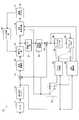•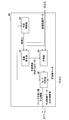•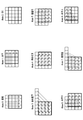•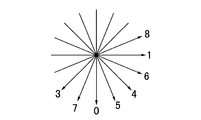•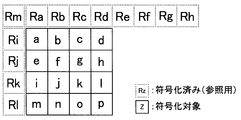•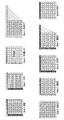•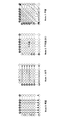•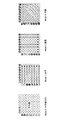••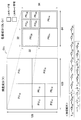•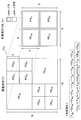•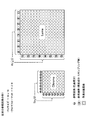•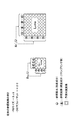•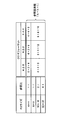•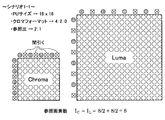•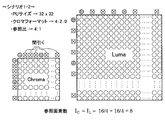•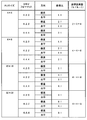•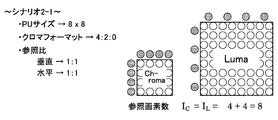•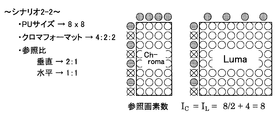•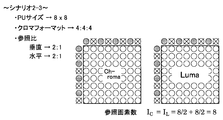•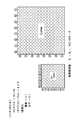•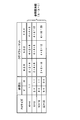•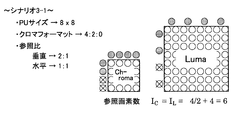•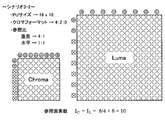•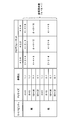•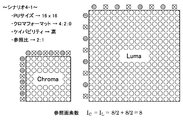•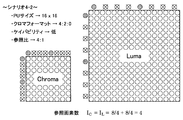•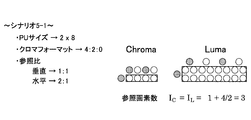•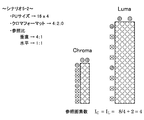•••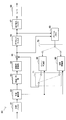•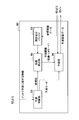•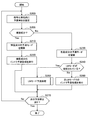•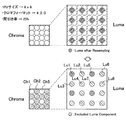•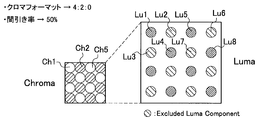•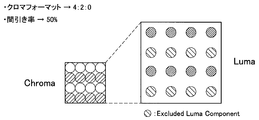•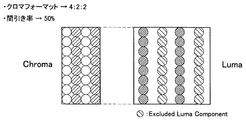•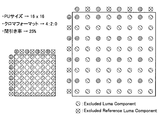•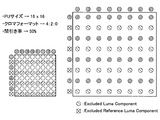•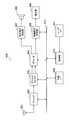••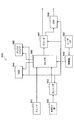•## Classifications

• HELECTRICITY
• H04ELECTRIC COMMUNICATION TECHNIQUE
• H04NPICTORIAL COMMUNICATION, e.g. TELEVISION
• H04N19/00Methods or arrangements for coding, decoding, compressing or decompressing digital video signals
• H04N19/10Methods or arrangements for coding, decoding, compressing or decompressing digital video signals using adaptive coding
• HELECTRICITY
• H04ELECTRIC COMMUNICATION TECHNIQUE
• H04NPICTORIAL COMMUNICATION, e.g. TELEVISION
• H04N19/00Methods or arrangements for coding, decoding, compressing or decompressing digital video signals
• H04N19/10Methods or arrangements for coding, decoding, compressing or decompressing digital video signals using adaptive coding
• H04N19/102Methods or arrangements for coding, decoding, compressing or decompressing digital video signals using adaptive coding characterised by the element, parameter or selection affected or controlled by the adaptive coding
• H04N19/103Selection of coding mode or of prediction mode
• H04N19/105Selection of the reference unit for prediction within a chosen coding or prediction mode, e.g. adaptive choice of position and number of pixels used for prediction
• HELECTRICITY
• H04ELECTRIC COMMUNICATION TECHNIQUE
• H04NPICTORIAL COMMUNICATION, e.g. TELEVISION
• H04N19/00Methods or arrangements for coding, decoding, compressing or decompressing digital video signals
• H04N19/10Methods or arrangements for coding, decoding, compressing or decompressing digital video signals using adaptive coding
• H04N19/102Methods or arrangements for coding, decoding, compressing or decompressing digital video signals using adaptive coding characterised by the element, parameter or selection affected or controlled by the adaptive coding
• H04N19/103Selection of coding mode or of prediction mode
• H04N19/11Selection of coding mode or of prediction mode among a plurality of spatial predictive coding modes
• HELECTRICITY
• H04ELECTRIC COMMUNICATION TECHNIQUE
• H04NPICTORIAL COMMUNICATION, e.g. TELEVISION
• H04N19/00Methods or arrangements for coding, decoding, compressing or decompressing digital video signals
• H04N19/10Methods or arrangements for coding, decoding, compressing or decompressing digital video signals using adaptive coding
• H04N19/134Methods or arrangements for coding, decoding, compressing or decompressing digital video signals using adaptive coding characterised by the element, parameter or criterion affecting or controlling the adaptive coding
• H04N19/157Assigned coding mode, i.e. the coding mode being predefined or preselected to be further used for selection of another element or parameter
• HELECTRICITY
• H04ELECTRIC COMMUNICATION TECHNIQUE
• H04NPICTORIAL COMMUNICATION, e.g. TELEVISION
• H04N19/00Methods or arrangements for coding, decoding, compressing or decompressing digital video signals
• H04N19/10Methods or arrangements for coding, decoding, compressing or decompressing digital video signals using adaptive coding
• H04N19/169Methods or arrangements for coding, decoding, compressing or decompressing digital video signals using adaptive coding characterised by the coding unit, i.e. the structural portion or semantic portion of the video signal being the object or the subject of the adaptive coding
• H04N19/186Methods or arrangements for coding, decoding, compressing or decompressing digital video signals using adaptive coding characterised by the coding unit, i.e. the structural portion or semantic portion of the video signal being the object or the subject of the adaptive coding the unit being a colour or a chrominance component
• HELECTRICITY
• H04ELECTRIC COMMUNICATION TECHNIQUE
• H04NPICTORIAL COMMUNICATION, e.g. TELEVISION
• H04N19/00Methods or arrangements for coding, decoding, compressing or decompressing digital video signals
• H04N19/50Methods or arrangements for coding, decoding, compressing or decompressing digital video signals using predictive coding
• H04N19/593Methods or arrangements for coding, decoding, compressing or decompressing digital video signals using predictive coding involving spatial prediction techniques
• HELECTRICITY
• H04ELECTRIC COMMUNICATION TECHNIQUE
• H04NPICTORIAL COMMUNICATION, e.g. TELEVISION
• H04N19/00Methods or arrangements for coding, decoding, compressing or decompressing digital video signals
• H04N19/44Decoders specially adapted therefor, e.g. video decoders which are asymmetric with respect to the encoder

## Abstract

Provided is an image processing device provided with a decoding unit that, in order to reduce the amount of memory resources required when performing intra-prediction that is on the basis of a prediction function that is dynamically constructed, decodes the luma and chroma of a block within an encoding unit in the order of the luma and the chroma of each block. The image processing device can typically be realized as an image decoding device that decodes an image. Also, provided is an image processing device provided with an encoding unit that encodes the luma and chroma of a block in an encoding unit in the order of the luma and the chroma of each block. The image processing device can typically be realized as an image encoding device that encodes an image.

## Description

Image processing apparatus and image processing method

The present disclosure relates to an image processing apparatus and an image processing method.

Conventionally, compression is intended to efficiently transmit or store digital images, and compresses the amount of information of an image using orthogonal transform such as discrete cosine transform and motion compensation, for example, using redundancy unique to the image. Technology is widespread. For example, H.264 developed by ITU-T. Image encoding devices and image decoding devices compliant with standard technologies such as the 26x standard or the MPEG-y standard established by the Moving Picture Experts Group (MPEG), store and distribute images by broadcast stations, and receive images by general users It is widely used in various situations such as storage.

H. The 26x standard (ITU-T Q6 / 16 VCEG) is a standard originally developed for the purpose of encoding suitable for communication applications such as videophone or videoconferencing. H. The 26x standard is known to be able to realize a higher compression ratio while requiring a larger amount of calculation for encoding and decoding than the MPEG-y standard. In addition, Joint Model of Enhanced-Compression Video Coding as part of MPEG4 activities Based on the 26x standard, a standard that can achieve a higher compression ratio has been established by incorporating new functions. This standard was approved in March 2003 by H.264. H.264 and MPEG-4 Part 10 (Advanced Video Coding; AVC) have become international standards.

One of the important techniques in the above-described image coding method is intra-screen prediction, that is, intra prediction. Intra prediction is a technique for reducing the amount of encoded information by using the correlation between adjacent blocks in an image and predicting the pixel values in a block from the pixel values of other adjacent blocks. . In the image coding system before MPEG4, only the DC component and low-frequency component of the orthogonal transform coefficient are targeted for intra prediction. In H.264 / AVC, intra prediction is possible for all pixel values. By using intra prediction, for example, an image with a gradual change in pixel value, such as an image of a blue sky, is expected to greatly improve the compression ratio.

H. In H.264 / AVC, for example, intra prediction may be performed using a block of 4 × 4 pixels, 8 × 8 pixels, or 16 × 16 pixels as one processing unit (ie, prediction unit (PU)). H. In HEVC (High Efficiency Video Coding), which is being standardized as the next-generation image encoding method following H.264 / AVC, the size of the prediction unit is about to be expanded to 32 × 32 pixels and 64 × 64 pixels. (See Non-Patent Document 1 below).

In intra prediction, usually, an optimal prediction mode for predicting a pixel value of a prediction target block is selected from a plurality of prediction modes. The prediction mode can typically be distinguished by the prediction direction from the reference pixel to the prediction target pixel. For example, H.M. In H.264 / AVC, when predicting a color difference component, four prediction modes of average value prediction, horizontal prediction, vertical prediction, and plane prediction can be selected. Further, in HEVC, an additional prediction mode called a linear model (LM) mode is proposed in which a pixel function of a color difference component is predicted using a linear function of a luminance component that is dynamically constructed as a prediction function. (See Non-Patent Document 2 below).

Sung-Chang Lim, Hahyun Lee, et al. "Intra coding using extended block size" (VCEG-AL28, July 2009) Jianle Chen, et al. "CE6.a.4: Chroma intra prediction by reconstructed luma samples" (JCTVC-E266, March 2011)

However, in the method described in Non-Patent Document 2, the memory resource required to construct the LM mode prediction function increases as the number of reference pixels increases. In particular, in the HEVC in which the size of the prediction unit is expanded to 64 × 64 pixels at the maximum, it becomes necessary to prepare a large-scale memory for adopting the LM mode, which leads to downsizing of hardware or low cost. It can be an obstacle to crystallization.

Therefore, it is desirable to provide a method capable of reducing the amount of memory resources required when intra prediction based on a dynamically constructed prediction function is performed as in the LM mode.

According to the present disclosure, there is provided an image processing apparatus including a decoding unit that decodes a luminance component and a color difference component of a block in a coding unit in order of the luminance component and the color difference component for each block.

The image processing apparatus can typically be realized as an image decoding apparatus that decodes an image.

Further, according to the present disclosure, there is provided an image processing method including decoding the luminance component and the color difference component of a block in a coding unit in order of the luminance component and the color difference component for each block.

Further, according to the present disclosure, there is provided an image processing device including an encoding unit that encodes the luminance component and the color difference component of a block in an encoding unit in order of the luminance component and the color difference component for each block. .

The image processing apparatus can typically be realized as an image encoding apparatus that encodes an image.

Also, according to the present disclosure, there is provided an image processing method including encoding a luminance component and a color difference component of a block in an encoding unit in order of the luminance component and the color difference component for each block.

The technique according to the present disclosure can reduce the amount of memory resources required when intra prediction based on a dynamically constructed prediction function is performed.

It is a block diagram which shows an example of a structure of the image coding apparatus which concerns on one Embodiment. It is a block diagram which shows an example of a detailed structure of the intra estimation part of the image coding apparatus which concerns on one Embodiment. It is explanatory drawing for demonstrating the example of the candidate of the prediction mode about the luminance component of the prediction unit of 4x4 pixel. It is explanatory drawing for demonstrating the prediction direction relevant to the example of FIG. It is explanatory drawing for demonstrating the reference pixel relevant to the example of FIG. It is explanatory drawing for demonstrating the example of the candidate of the prediction mode about the luminance component of a prediction unit of 8x8 pixel. It is explanatory drawing for demonstrating the example of the candidate of a prediction mode about the luminance component of a prediction unit of 16x16 pixel. It is explanatory drawing for demonstrating the example of the candidate of the prediction mode about a color difference component. It is explanatory drawing for demonstrating the difference between the order of the process in the existing method, and the order of the process in this embodiment. It is explanatory drawing for demonstrating the 1st example of the order of a new encoding process. It is explanatory drawing for demonstrating the 2nd example of the order of a new encoding process. It is the 1st explanatory view for explaining the reference pixel in LM mode. It is the 2nd explanatory view for explaining the reference pixel in LM mode. It is explanatory drawing which shows an example of the definition of the reference ratio in a 1st scenario. It is explanatory drawing which shows the 1st example of the reference pixel number controlled according to a 1st scenario. It is explanatory drawing which shows the 2nd example of the reference pixel number controlled according to a 1st scenario. It is explanatory drawing which shows an example of the definition of the reference ratio in a 2nd scenario. It is explanatory drawing which shows the 1st example of the reference pixel number controlled according to a 2nd scenario. It is explanatory drawing which shows the 2nd example of the reference pixel number controlled according to a 2nd scenario. It is explanatory drawing which shows the 3rd example of the reference pixel number controlled according to a 2nd scenario. It is explanatory drawing which shows the 4th example of the number of reference pixels controlled according to a 2nd scenario. It is explanatory drawing which shows an example of the definition of the reference ratio in a 3rd scenario. It is explanatory drawing which shows the 1st example of the reference pixel number controlled according to a 3rd scenario. It is explanatory drawing which shows the 2nd example of the reference pixel number controlled according to a 3rd scenario. It is explanatory drawing which shows an example of the definition of the reference ratio in a 4th scenario. It is explanatory drawing which shows the 1st example of the reference pixel number controlled according to a 4th scenario. It is explanatory drawing which shows the 2nd example of the reference pixel number controlled according to a 4th scenario. It is explanatory drawing which shows the 1st example of the reference pixel number controlled according to a 5th scenario. It is explanatory drawing which shows the 2nd example of the reference pixel number controlled according to a 5th scenario. It is a flowchart which shows an example of the flow of the intra prediction process at the time of the encoding which concerns on one Embodiment. It is a flowchart which shows an example of the detailed flow of the LM mode prediction process of FIG. It is a block diagram which shows an example of a structure of the image decoding apparatus which concerns on one Embodiment. It is a block diagram which shows an example of a detailed structure of the intra estimation part of the image decoding apparatus which concerns on one Embodiment. It is a flowchart which shows an example of the flow of the intra prediction process at the time of the decoding which concerns on one Embodiment. It is explanatory drawing for demonstrating an example of the thinning-out process which concerns on one modification. FIG. 27 is a first explanatory diagram for explaining a thinning rate different from the example of FIG. 26. FIG. 27 is a second explanatory diagram for explaining a thinning rate different from the example of FIG. 26. FIG. 27 is a third explanatory diagram for describing a thinning rate different from the example of FIG. 26. It is explanatory drawing for demonstrating the 1st example of a response | compatibility with the thinning position of a reference pixel, and the thinning position of a luminance component. It is explanatory drawing for demonstrating the 2nd example of a response | compatibility with the thinning position of a reference pixel, and the thinning position of a luminance component. It is a block diagram which shows an example of a schematic structure of a television apparatus. It is a block diagram which shows an example of a schematic structure of a mobile telephone. It is a block diagram which shows an example of a schematic structure of a recording / reproducing apparatus. It is a block diagram which shows an example of a schematic structure of an imaging device.

Hereinafter, preferred embodiments of the present disclosure will be described in detail with reference to the accompanying drawings. In addition, in this specification and drawing, about the component which has the substantially same function structure, duplication description is abbreviate | omitted by attaching | subjecting the same code | symbol.

The description will be given in the following order.
1. 1. Configuration example of image encoding device according to one embodiment 2. Processing flow during encoding according to an embodiment 3. Configuration example of image decoding apparatus according to one embodiment 4. Process flow during decoding according to one embodiment Modification 6 Application example 7. Summary

<1. Configuration Example of Image Encoding Device According to One Embodiment>
[1-1. Overall configuration example]
FIG. 1 is a block diagram illustrating an example of a configuration of an image encoding device 10 according to an embodiment. Referring to FIG. 1, an image encoding device 10 includes an A / D (Analogue to Digital) conversion unit 11, a rearrangement buffer 12, a subtraction unit 13, an orthogonal transformation unit 14, a quantization unit 15, a lossless encoding unit 16, The accumulation buffer 17, rate control unit 18, inverse quantization unit 21, inverse orthogonal transform unit 22, addition unit 23, deblock filter 24, frame memory 25, selectors 26 and 27, motion search unit 30, and intra prediction unit 40 Prepare.

The A / D converter 11 converts an image signal input in an analog format into image data in a digital format, and outputs a series of digital image data to the rearrangement buffer 12.

The rearrangement buffer 12 rearranges images included in a series of image data input from the A / D conversion unit 11. The rearrangement buffer 12 rearranges the images according to the GOP (Group of Pictures) structure related to the encoding process, and then outputs the rearranged image data to the subtraction unit 13, the motion search unit 30, and the intra prediction unit 40. To do.

The subtraction unit 13 is supplied with image data input from the rearrangement buffer 12 and predicted image data input from the motion search unit 30 or the intra prediction unit 40 described later. The subtraction unit 13 calculates prediction error data that is the difference between the image data input from the rearrangement buffer 12 and the predicted image data, and outputs the calculated prediction error data to the orthogonal transform unit 14.

The orthogonal transform unit 14 performs orthogonal transform on the prediction error data input from the subtraction unit 13. The orthogonal transformation performed by the orthogonal transformation part 14 may be discrete cosine transformation (Discrete Cosine Transform: DCT) or Karoonen-Labe transformation, for example. The orthogonal transform unit 14 outputs transform coefficient data acquired by the orthogonal transform process to the quantization unit 15.

The quantization unit 15 is supplied with transform coefficient data input from the orthogonal transform unit 14 and a rate control signal from the rate control unit 18 described later. The quantizing unit 15 quantizes the transform coefficient data and outputs the quantized transform coefficient data (hereinafter referred to as quantized data) to the lossless encoding unit 16 and the inverse quantization unit 21. Further, the quantization unit 15 changes the bit rate of the quantized data input to the lossless encoding unit 16 by switching the quantization parameter (quantization scale) based on the rate control signal from the rate control unit 18. Let

The lossless encoding unit 16 generates an encoded stream by performing a lossless encoding process on the quantized data input from the quantization unit 15. The lossless encoding by the lossless encoding unit 16 may be variable length encoding or arithmetic encoding, for example. Further, the lossless encoding unit 16 multiplexes information related to intra prediction or information related to inter prediction input from the selector 27 in the header region of the encoded stream. Then, the lossless encoding unit 16 outputs the generated encoded stream to the accumulation buffer 17.

Usually, one coding unit (CU) includes one or more prediction units for the luminance component (Y) and one or more prediction units for each of the color difference components (Cb, Cr). In the existing method, the quantized data of these prediction units is encoded in the order of each component. That is, after the luminance component (Y) data in one CU is encoded, the color difference component (Cb) data is encoded, and then the color difference component (Cr) data is encoded. On the other hand, in this embodiment, the lossless encoding part 16 encodes quantized data in the order according to PU about the encoding unit in which intra prediction is performed, and produces | generates an encoding stream. The order of such encoding will be further described later.

The accumulation buffer 17 temporarily accumulates the encoded stream input from the lossless encoding unit 16 using a storage medium such as a semiconductor memory. Then, the accumulation buffer 17 outputs the accumulated encoded stream to a transmission unit (not shown) (for example, a communication interface or a connection interface with a peripheral device) at a rate corresponding to the bandwidth of the transmission path.

The rate control unit 18 monitors the free capacity of the accumulation buffer 17. Then, the rate control unit 18 generates a rate control signal according to the free capacity of the accumulation buffer 17 and outputs the generated rate control signal to the quantization unit 15. For example, the rate control unit 18 generates a rate control signal for reducing the bit rate of the quantized data when the free capacity of the storage buffer 17 is small. For example, when the free capacity of the accumulation buffer 17 is sufficiently large, the rate control unit 18 generates a rate control signal for increasing the bit rate of the quantized data.

The inverse quantization unit 21 performs an inverse quantization process on the quantized data input from the quantization unit 15. Then, the inverse quantization unit 21 outputs transform coefficient data acquired by the inverse quantization process to the inverse orthogonal transform unit 22.

The inverse orthogonal transform unit 22 restores the prediction error data by performing an inverse orthogonal transform process on the transform coefficient data input from the inverse quantization unit 21. Then, the inverse orthogonal transform unit 22 outputs the restored prediction error data to the addition unit 23.

The adding unit 23 generates decoded image data by adding the restored prediction error data input from the inverse orthogonal transform unit 22 and the predicted image data input from the motion search unit 30 or the intra prediction unit 40. . Then, the addition unit 23 outputs the generated decoded image data to the deblock filter 24 and the frame memory 25.

The deblocking filter 24 performs a filtering process for reducing block distortion that occurs during image coding. The deblocking filter 24 removes block distortion by filtering the decoded image data input from the adding unit 23, and outputs the decoded image data after filtering to the frame memory 25.

The frame memory 25 stores the decoded image data input from the adder 23 and the decoded image data after filtering input from the deblock filter 24 using a storage medium.

The selector 26 reads out the decoded image data after filtering used for inter prediction from the frame memory 25 and supplies the read out decoded image data to the motion search unit 30 as reference image data. The selector 26 reads out decoded image data before filtering used for intra prediction from the frame memory 25 and supplies the read decoded image data to the intra prediction unit 40 as reference image data.

In the inter prediction mode, the selector 27 outputs the prediction image data as a result of the inter prediction output from the motion search unit 30 to the subtraction unit 13 and outputs information related to the inter prediction to the lossless encoding unit 16. Further, in the intra prediction mode, the selector 27 outputs predicted image data as a result of the intra prediction output from the intra prediction unit 40 to the subtraction unit 13 and outputs information related to the intra prediction to the lossless encoding unit 16. . The selector 27 switches between the inter prediction mode and the intra prediction mode according to the size of the cost function value output from the motion search unit 30 and the intra prediction unit 40.

The motion search unit 30 performs inter prediction processing (interframe prediction processing) based on the image data to be encoded (original image data) input from the rearrangement buffer 12 and the decoded image data supplied via the selector 26. )I do. For example, the motion search unit 30 evaluates the prediction result in each prediction mode using a predetermined cost function. Next, the motion search unit 30 selects the prediction mode with the smallest cost function value, that is, the prediction mode with the highest compression rate, as the optimum prediction mode. In addition, the motion search unit 30 generates predicted image data according to the optimal prediction mode. Then, the motion search unit 30 outputs prediction mode information indicating the selected optimal prediction mode, information on inter prediction including motion vector information and reference image information, a cost function value, and predicted image data to the selector 27.

The intra prediction unit 40 performs an intra prediction process for each block set in the image based on the original image data input from the rearrangement buffer 12 and the decoded image data as reference image data supplied from the frame memory 25. I do. Then, the intra prediction unit 40 outputs information related to intra prediction including the prediction mode information indicating the optimal prediction mode and the size related information, the cost function value, and the predicted image data to the selector 27. The prediction modes selectable by the intra prediction unit 40 include a linear model (LM) mode for color difference components in addition to the existing intra prediction modes. Unlike the LM mode described in Non-Patent Document 2, the LM mode in this embodiment has a feature that the ratio of the number of reference pixels to the block size can be changed. Such intra prediction processing by the intra prediction unit 40 will be described in detail later.

[1-2. Configuration example of intra prediction unit]
FIG. 2 is a block diagram illustrating an example of a detailed configuration of the intra prediction unit 40 of the image encoding device 10 illustrated in FIG. 1. Referring to FIG. 2, the intra prediction unit 40 includes a prediction control unit 42, a coefficient calculation unit 44, a prediction unit 46, and a mode determination unit 48.

The prediction control unit 42 controls the intra prediction process in the intra prediction unit 40. For example, the prediction control unit 42 executes the intra prediction process for the luminance component (Y) in a certain processing unit, and then executes the intra prediction process for the color difference components (Cb, Cr). In the intra prediction process for the luminance component, the prediction control unit 42 causes the prediction unit 46 to generate a prediction pixel value of each pixel in a plurality of prediction modes, and causes the mode determination unit 48 to determine the optimal prediction mode of the luminance component. As a result, the arrangement of prediction units within the coding unit is also determined. In the intra prediction process for the color difference component, the prediction control unit 42 causes the prediction unit 46 to generate a prediction pixel value of each pixel in a plurality of prediction modes for each prediction unit, and causes the mode determination unit 48 to optimally predict the color difference component. To determine.

Prediction mode candidates for luminance components are H.264. It may be a prediction mode employed in an existing image coding method such as H.264 / AVC, and may include other prediction modes. Prediction mode candidates for color difference components may also include prediction modes employed in existing image coding schemes. Further, the prediction mode candidates for the color difference components include the LM mode described above. However, in the present embodiment, the LM mode is the prediction mode of the prediction unit of the color difference component only when the size of the prediction unit of the color difference component and the size of the prediction unit of the corresponding luminance component satisfy a predetermined condition. It is added to the candidate (ie, the search range of the optimal prediction mode). The predetermined condition may be a condition that “the size of the prediction unit of the color difference component is equal to or smaller than a size determined according to the chroma format from the size of the prediction unit of the corresponding luminance component”. Here, the “corresponding prediction unit” refers to a prediction unit that at least partially shares a pixel. The size of the prediction unit of the color difference component can be determined without depending on the size of the prediction unit of the luminance component. Therefore, for a certain color difference component prediction unit, there may be a corresponding one or more luminance component prediction units. The “size determined according to the chroma format from the size of the prediction unit of the corresponding luminance component” in the above condition means that the chroma format is 4: 2: 0 when the size of the prediction unit of the luminance component is M × N pixels. If there are (M / 2) × (N / 2) pixels, if the chroma format is 4: 2: 2, (M / 2) × N pixels, if the chroma format is 4: 4: 4, then M × N pixels. It is. The significance of such LM mode search limitations will be described in more detail later.

Further, in the method described in Non-Patent Document 2, the ratio of the number of reference pixels to the block size when calculating the coefficient of the prediction function in the LM mode is constant. Therefore, as the block size increases, the number of reference pixels increases accordingly. On the other hand, in the present embodiment, the prediction control unit 42 variably controls the ratio. The block size here means the size of the prediction unit in principle. In this specification, the above-described ratio controlled by the prediction control unit 42, that is, the ratio of the number of reference pixels to the block size is referred to as a “reference ratio”. The control of the reference ratio by the prediction control unit 42 can typically be performed according to the block size. Further, the prediction control unit 42 may control the reference ratio according to a chroma format that affects the block size of the color difference component. Further, the prediction control unit 42 may control the reference ratio according to a parameter (for example, a profile or a level) that defines the capability of the apparatus related to image encoding and decoding. A plurality of scenarios of reference ratio control by the prediction control unit 42 will be described in detail later.

The coefficient calculation unit 44 calculates the coefficient of the prediction function used by the prediction unit 46 in the LM mode with reference to the pixels around the prediction unit to which the prediction target pixel belongs, that is, the reference pixel. The prediction function used by the prediction unit 46 is typically a linear function of luminance component values. The number of reference pixels referred to by the coefficient calculation unit 44 when calculating the coefficient of the prediction function is controlled by the prediction control unit 42 as described above.

The prediction unit 46 predicts the pixel value of the luminance component and the pixel value of the color difference component of the pixel to be predicted according to various prediction mode candidates under the control of the prediction control unit 42. An example of prediction mode candidates used by the prediction unit 46 will be described in detail later. Predicted image data generated as a result of prediction by the prediction unit 46 is output to the mode determination unit 48 for each prediction mode.

The mode determination unit 48 calculates a cost function value for each prediction mode based on the original image data input from the rearrangement buffer 12 and the predicted image data input from the prediction unit 46. Then, the mode determination unit 48 determines the optimal prediction mode for the luminance component and the arrangement of the prediction unit in the coding unit based on the calculated cost function value. Similarly, the mode determination unit 48 determines the optimal prediction mode and the arrangement of prediction units for the color difference component based on the cost function value for the color difference component. Then, the mode determination unit 48 predicts image data including information related to intra prediction including prediction mode information indicating the determined optimal prediction mode and size related information, cost function values, and prediction pixel values of luminance components and color difference components. Is output to the selector 27. The size related information output from the mode determination unit 48 may include information specifying a chroma format in addition to information for specifying the size of each prediction unit.

[1-3. Prediction mode candidates]
Next, prediction mode candidates that can be used by the prediction unit 46 of the intra prediction unit 40 will be described.

(1) Prediction mode candidates for luminance components Prediction mode candidates for luminance components are The prediction mode may be a prediction mode employed in an existing image coding method such as H.264 / AVC. 3 to 5 are explanatory diagrams for explaining such prediction mode candidates when the size of the prediction unit is 4 × 4 pixels.

Referring to FIG. 3, nine types of prediction modes (mode 0 to mode 8) that can be used in a prediction unit of 4 × 4 pixels are shown. FIG. 4 schematically shows prediction directions corresponding to the mode numbers. In FIG. 5, lower case alphabets a to p represent pixel values of each pixel (that is, a prediction target pixel) in a prediction unit of 4 × 4 pixels. Rz (z = a, b,..., M) around the prediction unit represents the pixel value of the encoded reference pixel.

For example, the prediction direction in mode 0 is the vertical direction, and each prediction pixel value is calculated as follows:
a = e = i = m = Ra
b = f = j = n = Rb
c = g = k = o = Rc
d = h = l = p = Rd

The prediction direction in mode 1 is the horizontal direction, and each prediction pixel value is calculated as follows:
a = b = c = d = Ri
e = f = g = h = Rj
i = j = k = l = Rk
m = n = o = p = Rl

Mode 2 represents DC prediction (average prediction), and each predicted pixel value is calculated according to one of the following four formulas depending on which reference pixel is available:
a = b = ... = p = (Ra + Rb + Rc + Rd + Ri + Rj + Rk + Rl + 4) >> 3
a = b =... = p = (Ra + Rb + Rc + Rd + 2) >> 2
a = b = ... = p = (Ri + Rj + Rk + Rl + 2) >> 2
a = b =... = p = 128

The prediction direction in mode 3 is diagonally lower left, and each predicted pixel value is calculated as follows:
a = (Ra + 2Rb + Rc + 2) >> 2
b = e = (Rb + 2Rc + Rd + 2) >> 2
c = f = i = (Rc + 2Rd + Re + 2) >> 2
d = g = j = m = (Rd + 2Re + Rf + 2) >> 2
h = k = n = (Re + 2Rf + Rg + 2) >> 2
l = o = (Rf + 2Rg + Rh + 2) >> 2
p = (Rg + 3Rh + 2) >> 2

The prediction direction in mode 4 is diagonally lower right, and each predicted pixel value is calculated as follows:
m = (Rj + 2Rk + Rl + 2) >> 2
i = n = (Ri + 2Rj + Rk + 2) >> 2
e = j = o = (Rm + 2Ri + Rj + 2) >> 2
a = f = k = p = (Ra + 2Rm + Ri + 2) >> 2
b = g = 1 = (Rm + 2Ra + Rb + 2) >> 2
c = h = (Ra + 2Rb + Rc + 2) >> 2
d = (Rb + 2Rc + Rd + 2) >> 2

The prediction direction in mode 5 is vertical right and each predicted pixel value is calculated as follows:
a = j = (Rm + Ra + 1) >> 1
b = k = (Ra + Rb + 1) >> 1
c = l = (Rb + Rc + 1) >> 1
d = (Rc + Rd + 1) >> 1
e = n = (Ri + 2Rm + Ra + 2) >> 2
f = o = (Rm + 2Ra + Rb + 2) >> 2
g = p = (Ra + 2Rb + Rc + 2) >> 2
h = (Rb + 2Rc + Rd + 2) >> 2
i = (Rm + 2Ri + Rj + 2) >> 2
m = (Ri + 2Rj + Rk + 2) >> 2

The prediction direction in mode 6 is horizontal and each predicted pixel value is calculated as follows:
a = g = (Rm + Ri + 1) >> 1
b = h = (Ri + 2Rm + Ra + 2) >> 2
c = (Rm + 2Ra + Rb + 2) >> 2
d = (Ra + 2Rb + Rc + 2) >> 2
e = k = (Ri + Rj + 1) >> 1
f = l = (Rm + 2Ri + Rj + 2) >> 2
i = o = (Rj + Rk + 1) >> 1
j = p = (Ri + 2Rj + Rk + 2) >> 2
m = (Rk + Rl + 1) >> 1
n = (Rj + 2Rk + Rl + 2) >> 2

The prediction direction in mode 7 is vertical left, and each prediction pixel value is calculated as follows:
a = (Ra + Rb + 1) >> 1
b = i = (Rb + Rc + 1) >> 1
c = j = (Rc + Rd + 1) >> 1
d = k = (Rd + Re + 1) >> 1
l = (Re + Rf + 1) >> 1
e = (Ra + 2Rb + Rc + 2) >> 2
f = m = (Rb + 2Rc + Rd + 2) >> 2
g = n = (Rc + 2Rd + Re + 2) >> 2
h = o = (Rd + 2Re + Rf + 2) >> 2
p = (Re + 2Rf + Rg + 2) >> 2

The prediction direction in mode 8 is horizontal, and each predicted pixel value is calculated as follows:
a = (Ri + Rj + 1) >> 1
b = (Ri + 2Rj + Rk + 2) >> 2
c = e = (Rj + Rk + 1) >> 1
d = f = (Rj + 2Rk + Rl + 2) >> 2
g = i = (Rk + Rl + 1) >> 1
h = j = (Rk + 3Rl + 2) >> 2
k = l = m = n = o = p = Rl

Referring to FIG. 6, nine types of prediction modes (mode 0 to mode 8) that can be used in a prediction unit of 8 × 8 pixels are shown. The prediction direction in mode 0 is the vertical direction. The prediction direction in mode 1 is the horizontal direction. Mode 2 represents DC prediction (average value prediction). The prediction direction in mode 3 is diagonally lower left. The prediction direction in mode 4 is diagonally lower right. The prediction direction in mode 5 is vertical right. The prediction direction in mode 6 is horizontally below. The prediction direction in mode 7 is vertical left. The prediction direction in mode 8 is horizontal.

Referring to FIG. 7, four types of prediction modes (mode 0 to mode 3) that can be used in a prediction unit of 16 × 16 pixels are shown. The prediction direction in mode 0 is the vertical direction. The prediction direction in mode 1 is the horizontal direction. Mode 2 represents DC prediction (average value prediction). Mode 3 represents planar prediction.

(2) Candidate prediction modes for color difference components The prediction mode for color difference components can be selected independently of the prediction mode for luminance components. FIG. 8 shows a prediction mode among the prediction mode candidates that can be used when the block size of the color difference component is 8 × 8 pixels. Four prediction modes (mode 0 to mode 3) employed in existing image coding schemes such as H.264 / AVC are shown.

Mode 0 represents DC prediction (average value prediction). Here, the predicted pixel value at the pixel position (x, y) is Pr C (x, y), the left eight reference pixel values are Re C (−1, n), and the upper eight reference pixel values are Re C. Let (n, -1). Subscript C means a color difference component. n is an integer of 0 or more and 7 or less. The predicted pixel value Pr C (x, y) is then calculated according to one of the following three formulas, depending on which reference pixel is available:

The prediction direction in mode 1 is the horizontal direction, and the predicted pixel value Pr C (x, y) is calculated as follows:

The prediction direction in mode 2 is the vertical direction, and the predicted pixel value Pr C (x, y) is calculated as follows:

Mode 3 represents planar prediction. The predicted pixel value Pr C (x, y) is calculated as follows:

Furthermore, in this embodiment, the LM mode described in the next section can be selected (for example, as mode 4) for the color difference component.

[1-4. Details of LM mode]
In the LM mode, the predicted pixel value for the color difference component is calculated using a linear function of the value of the corresponding luminance component. For example, the prediction function used in the LM mode may be a linear linear function described in Non-Patent Document 2 as follows:

In the formula (1), Re L '( x, y) represents the value of the decoded image pixel positions after resampling of the luminance component (so-called re-construct the image) (x, y). The resampling of the luminance component is performed when the resolution of the color difference component differs from the resolution of the luminance component depending on the chroma format. For example, when the chroma format is 4: 2: 0, the luminance component is resampled according to the following equation so that the number of pixels is halved in both the horizontal direction and the vertical direction. Note that Re L (u, v) represents the value of the luminance component at the pixel position (u, v) before resampling.

When the chroma format is 4: 2: 2, the luminance component is resampled so that the number of pixels is halved in the horizontal direction. When the chroma format is 4: 4: 4, resampling of the luminance component is not performed.

The coefficient α in the equation (1) is calculated according to the following equation (3). Further, the coefficient β of the equation (1) can be calculated according to the following equation (4).

In Expressions (3) and (4), I represents the number of reference pixels. For example, if the block size of the color difference component is 8 × 8 pixels and the left eight reference pixels and the upper eight reference pixels are both available, I = 8 + 8 = 16.

[1-5. New encoding process order]
Expressions (1) to (4) described above are the same as the expressions described in Non-Patent Document 2. Re L ′ (x, y) on the right side of Expression (1) represents the value of the pixel position (x, y) after the re-sampling of the luminance component of the decoded image (the reconstructed image in the encoder or the decoded image in the decoder). . Therefore, when the predicted pixel value is calculated in units of blocks, the pixel value after resampling of the luminance component of the corresponding block is at least temporarily held until the predicted pixel value for the color difference component is calculated. Need to be kept.

FIG. 9 is an explanatory diagram for explaining the difference between the order of processing in the existing method and the order of processing in the present embodiment. Referring to FIG. 9, LCU 1 including coding units CU 0 , CU 1 , CU 2 and other coding units is illustrated as an example. Furthermore, the coding unit CU 0 is divided into four prediction units PU 00 , PU 01 , PU 02 and PU 03 . The coding unit CU 1 is divided into four prediction units PU 10 , PU 11 , PU 12 and PU 13 . The coding unit CU 2 is divided into four prediction units PU 20 , PU 21 , PU 22 and PU 23 . Here, for the sake of simplicity of explanation, it is assumed that the prediction units of all the color difference components have sizes determined according to the chroma format from the sizes of the prediction units of the corresponding luminance components. In other words, the luminance component prediction unit and the color difference component prediction unit have a one-to-one correspondence. In the existing method, the image data encoding process for such an LCU is performed as follows: Y 00 → Y 01 → Y 02 → Y 03 → Cb 00 → Cb 01 → Cb 02 → Cb 03 → Cr 00 → Cr 01 → Cr It is performed in the order of 02 → Cr 03 → Y 10 →. Here, Y NN represents the encoding process of the luminance component of the prediction unit PU NN , and Cb NN and Cr NN represent the encoding process of the color difference component of the prediction unit PU NN , respectively. The same applies to the intra prediction process. That is, in the existing method, encoding processing is performed for each component for each encoding unit. In this specification, such an order of processing is referred to as “component order”. On the other hand, in the present embodiment, image data encoding processing is performed for each prediction unit for each encoding unit. In this specification, such a new processing order is referred to as “order by PU”. For example, when the order by PU is applied to LCU 1 in FIG. 9, first , the encoding process of the luminance component Y 00 and the two color difference components Cb 00 and Cr 00 for the prediction unit PU 00 is performed. Next, encoding processing of the luminance component Y 01 and the two color difference components Cb 01 and Cr 01 for the prediction unit PU 01 is performed. Thereafter, the encoding process of the three components is repeated in the order of the prediction units PU 02 , PU 03 , PU 10 .

In the order by component, which is an existing method, the required amount of memory resources for holding the value of the luminance component referenced in the intra prediction in the LM mode depends on the size of the maximum coding unit. For example, if the maximum coding unit size is 128 × 128 pixels, the chroma format is 4: 2: 0, and the bit depth is 10 bits, a memory resource of 64 × 64 × 10 bits can be consumed. On the other hand, in the above-described order by PU, the required amount of memory resources depends on the size of the maximum prediction unit of the color difference component. In HEVC, the size of the maximum prediction unit of the luminance component is 64 × 64 pixels. Therefore, when the above-described order by PU is adopted, if the chroma format is 4: 2: 0 and the bit depth is 10 bits, the required amount of memory resources is at most 32 × 32 × 10 bits. .

Furthermore, in the present embodiment, as described above, a limit is imposed on the search in the LM mode. That is, only when the size of the prediction unit of the color difference component is equal to or smaller than the size determined according to the chroma format from the size of the prediction unit of the corresponding luminance component, the LM mode is a prediction mode candidate for the prediction unit of the color difference component. Added to. As a result, in the case where the LM mode is selected, only one prediction unit for one luminance component corresponds to one prediction unit for a color difference component. Accordingly, when the order by PU is adopted, when the processing shifts from the prediction unit of the first luminance component to the prediction unit of the second luminance component, in principle, the prediction unit of the first luminance component is buffered. Data can be cleared.

Considering the significance of the limitation of the search in the LM mode, generally, in a case where a certain block is divided into a plurality of prediction units, the correlation of images between the plurality of prediction units is low. Even if the prediction function is determined based on the value of the luminance component over a plurality of luminance component prediction units with low correlation to each other, the prediction function can model the correlation between the luminance component and the color difference component with sufficient accuracy. It is thought that it is not. Therefore, even if the above-mentioned restrictions are imposed on the application of the LM mode, a prediction mode that cannot be expected to have high prediction accuracy is only excluded from the prediction mode candidates, so there is no significant disadvantage, but rather a reduction in processing cost. Profits are obtained.

If the luminance component prediction unit size is 4 × 4 pixels and the chroma format is 4: 2: 0, the color difference component prediction unit size determined in accordance with the chroma format is 2 × 2 pixels. is there. However, in HEVC, a 2 × 2 pixel prediction unit is not available. On the other hand, it has been reported that the LM mode contributes more greatly to the improvement in coding efficiency as the size of the prediction unit is smaller. Therefore, it is also beneficial to allow the application of the LM mode based on a plurality of prediction units of luminance components exceptionally only when the size of the prediction unit of luminance components is small (for example, 4 × 4 pixels). In this case, the lossless encoding unit 16 continuously performs a plurality of luminance component prediction units (for example, four 4 × 4 pixel prediction units) corresponding to one color difference component prediction unit (for example, 4 × 4 pixels). After the encoding is performed, the prediction unit of the one color difference component can be encoded.

FIG. 10A is an explanatory diagram for describing a first example of the order of new encoding processing that can be employed in the present embodiment. The left side of the figure shows an arrangement of luminance component prediction units (hereinafter referred to as luminance prediction units) in the coding unit CU 1 . Right in the drawing, the prediction unit of the color difference components of the coding unit CU 1 (hereinafter, referred to as color difference prediction units) shows the arrangement of the. The size of the coding unit CU 1 is 128 × 128 pixels, and the chroma format is 4: 2: 0. The coding unit CU 1 includes seven luminance prediction units PU 10 to PU 16 . The size of the luminance prediction units PU 10 , PU 11 and PU 16 is 64 × 64 pixels, and the size of the luminance prediction units PU 12 to PU 15 is 32 × 32 pixels. The coding unit CU 1 includes seven color difference prediction units PU 20 to PU 26 . The size of the color difference prediction unit PU 20 is 32 × 32 pixels, and the color difference prediction unit PU 20 corresponds to the luminance prediction unit PU 10 . The size of the color difference prediction units PU 21 to PU 24 is 16 × 16 pixels, and the color difference prediction units PU 21 to PU 24 correspond to the luminance prediction unit PU 11 . The size of the color difference prediction unit PU 25 is 32 × 32 pixels, and the color difference prediction unit PU 25 corresponds to the luminance prediction units PU 12 to PU 15 . The size of the color difference prediction unit PU 26 is 32 × 32 pixels, and the color difference prediction unit PU 26 corresponds to the luminance prediction unit PU 16 . Among these color difference prediction units PU 20 to PU 26 , the size of the color difference prediction unit PU 25 (32 × 32 pixels) is determined according to the chroma format from the size of the corresponding luminance prediction units PU 21 to PU 24 (32 × 32 pixels). It is larger than a fixed size (16 × 16 pixels). Therefore, for the color difference prediction unit PU 25 , the LM mode is excluded from the prediction mode candidates. On the other hand, for other color difference prediction units, the LM mode is not excluded from the prediction mode candidates.

In the example of FIG. 10A, the encoding process is as follows: Y 10 → Cb 20 → Cr 20 → Y 11 → Cb 21 → Cr 21 → Cb 22 → Cr 22 → Cb 23 → Cr 23 → Cb 24 → Cr 24 → Y 12 → It is performed in the order of Y 13 → Y 14 → Y 15 → Cb 25 → Cr 25 → Y 16 → Cb 26 → Cr 26 . That is, for example, after the luminance prediction unit PU 10 is encoded, the color difference prediction unit PU 20 is encoded before the luminance prediction unit PU 11 is encoded. In addition, after the luminance prediction unit PU 11 is encoded, the color difference prediction units PU 21 to PU 24 are encoded before the luminance prediction unit PU 12 is encoded. Therefore, even if the buffer size is 32 × 32 pixels, the resampled value of the luminance component of the corresponding luminance prediction unit at the time of intra prediction for each color difference prediction unit is not yet cleared. The LM mode can be used.

FIG. 10B is an explanatory diagram for describing a second example of the order of new encoding processing that can be employed in the present embodiment. Left in FIG shows an arrangement of luminance prediction unit of the coding unit in the CU 2. Right in the drawing shows the arrangement of the color difference prediction unit of the coding unit in the CU 2. The size of the coding unit CU 2 is 16 × 16 pixels, the chroma format is 4: 2: is assumed to be 0. The coding unit CU 2 includes 10 luminance prediction units PU 30 to PU 39 . The size of the luminance prediction units PU 30 and PU 39 is 8 × 8 pixels, and the size of the luminance prediction units PU 31 to PU 38 is 4 × 4 pixels. The coding unit CU 2 includes four color difference prediction units PU 40 to PU 43 . The size of the color difference prediction units PU 40 to PU 43 is 4 × 4 pixels, which is the smallest size available for the color difference prediction unit. The color difference prediction unit PU 40 is a luminance prediction unit PU 30 , the color difference prediction unit PU 41 is a luminance prediction unit PU 31 to PU 34 , the color difference prediction unit PU 42 is a luminance prediction unit PU 35 to PU 38 , and a color difference prediction unit PU 43. Corresponds to the luminance prediction unit PU 39 , respectively. The sizes (4 × 4 pixels) of the color difference prediction units PU 41 and PU 42 are determined according to the chroma format from the sizes (4 × 4 pixels) of the corresponding luminance prediction units PU 31 to PU 34 and PU 35 to PU 38. It is larger than (2 × 2 pixels). However, since the size of the corresponding luminance prediction unit is 4 × 4 pixels, the use of the LM mode can be permitted for the color difference prediction units PU 41 and PU 42 . Therefore, in the example of FIG. 10B, the LM mode can be added to the search target for all of the color difference prediction units PU 40 to PU 43 .

In the example of FIG. 10B, the encoding process is as follows: Y 30 → Cb 40 → Cr 40 → Y 31 → Y 32 → Y 33 → Y 34 → Cb 41 → Cr 41 → Y 35 → Y 36 → Y 37 → Y 38 → It is performed in the order of Cb 42 → Cr 42 → Y 39 → Cb 43 → Cr 43 . That is, for example, before the color difference prediction unit PU 41 is encoded, the luminance prediction units PU 31 to PU 34 are encoded. Therefore, intra prediction can be performed in the LM mode for the color difference prediction unit PU 41 based on the luminance component values of the four 4 × 4 pixel luminance prediction units PU 31 to PU 34 . Similarly, the luminance prediction units PU 35 to PU 38 are encoded before the color difference prediction unit PU 42 is encoded. Therefore, intra prediction can be performed in the LM mode for the color difference prediction unit PU 42 based on the luminance component values of the four 4 × 4 pixel luminance prediction units PU 35 to PU 38 .

[1-6. Control of the number of reference pixels]
11A and 11B are explanatory diagrams for further explaining the reference pixel in the LM mode.

In the example of FIG. 11A, the size of the prediction unit (PU) is 16 × 16 pixels, and the chroma format is 4: 2: 0. In this case, the block size of the color difference component is 8 × 8 pixels. The number of chrominance component reference pixels Re C (i) is 8 + 8 = 16 (assuming both the left and top reference pixels are available). The number of luminance component reference pixels Re L ′ (i) is also 8 + 8 = 16 as a result of resampling.

In the example of FIG. 11B, the size of the prediction unit (PU) is 8 × 8 pixels, and the chroma format is 4: 2: 0. In this case, the block size of the color difference component is 4 × 4 pixels. The number of reference pixels Re C (i) of the color difference component is 4 + 4 = 8. The number of reference pixels Re L ′ (i) in the luminance component is also 4 + 4 = 8 as a result of resampling.

11A and 11B are compared, if other conditions such as chroma format are the same, the ratio of the number of reference pixels to the block size has not changed. That is, in the example of FIG. 11A, the size of one side of the prediction unit is 16 pixels and the reference pixel number I is 16, whereas in the example of FIG. 11B, the size of one side of the prediction unit is 8 pixels. The number I is 8. Thus, if the number of reference pixels I increases as the block size increases, the processing cost required to calculate the coefficients α and β using the above equations (3) and (4) also increases. In particular, as can be understood by paying attention to Equation (3), the number of pixel value multiplications increases on the order of the square of the reference pixel number I. Therefore, if the number of reference pixels is not appropriately controlled when calculating the coefficient α and the coefficient β, there is a high possibility that a large amount of memory resources will be consumed when the block size is large. Therefore, the prediction control unit 42 variably controls the number of reference pixels when the coefficient calculation unit 44 calculates the coefficient α and the coefficient β of the prediction function in the LM mode.

Typically, the prediction control unit 42 controls the reference ratio, which is the ratio of the number of reference pixels to the block size, to be smaller as the block size is larger. Thereby, an increase in processing cost when the block size is increased is suppressed. The prediction control unit 42 may not change the reference ratio even if the block size is different when the block size is small enough that the processing cost does not become a problem. Hereinafter, five exemplary scenarios of reference ratio control will be described with reference to FIGS. 12 to 20B.

(1) First Scenario FIG. 12 is an explanatory diagram showing an example of the definition of the reference ratio in the first scenario.

In the first scenario, when the size of the prediction unit (PU) is 4 × 4 pixels, the reference ratio is “1: 1”. The reference ratio “1: 1” means that all the reference pixels as shown in FIG. 11A or FIG. 11B are used. In this case, if the chroma format is 4: 2: 0, the number of reference pixels I is 2 (vertical direction I v ) +2 (horizontal direction I h ) = 4. If the chroma format is 4: 2: 2, the reference pixel number I is 4 + 2 = 6. If the chroma format is 4: 4: 4, the reference pixel number I is 4 + 4 = 8.

Similarly, when the size of the prediction unit is 8 × 8 pixels, the reference ratio is “1: 1”. In this case, if the chroma format is 4: 2: 0, the reference pixel number I is 4 + 4 = 8. If the chroma format is 4: 2: 2, the reference pixel number I is 8 + 4 = 12. If the chroma format is 4: 4: 4, the reference pixel number I is 8 + 8 = 16.

On the other hand, when the size of the prediction unit is 16 × 16 pixels, the reference ratio is “2: 1”. The reference ratio “2: 1” means that only half of the reference pixels shown in FIG. 11A or 11B are used. That is, the coefficient calculation unit 44 uses only the remaining reference pixels after thinning out half of the reference pixels when calculating the coefficients α and β. In this case, if the chroma format is 4: 2: 0, the reference pixel number I is (8/2) + (8/2) = 8. If the chroma format is 4: 2: 2, the reference pixel number I is (16/2) + (8/2) = 12. If the chroma format is 4: 4: 4, the number of reference pixels I is (16/2) + (16/2) = 16.

FIG. 13A shows an example of reference pixel settings when the PU size is 16 × 16 pixels and the chroma format is 4: 2: 0. In the example of FIG. 13A, every other reference pixel of the color difference component and reference pixel of the luminance component are thinned out. As a result, the reference pixel number I L of the reference pixel number I C and the luminance component of the color difference component are both eight.

Furthermore, when the size of the prediction unit is 32 × 32 pixels, the reference ratio is “4: 1”. The reference ratio “4: 1” means that only one-fourth of the reference pixels as shown in FIG. 11A or 11B are used. That is, the coefficient calculation unit 44 uses only the remaining reference pixels after thinning out the three-fourth reference pixels when calculating the coefficients α and β. In this case, if the chroma format is 4: 2: 0, the reference pixel number I is (16/4) + (16/4) = 8. If the chroma format is 4: 2: 2, the reference pixel number I is (32/4) + (16/4) = 12. If the chroma format is 4: 4: 4, the reference pixel number I is (32/4) + (32/4) = 16.

FIG. 13B shows an example of reference pixel settings when the PU size is 32 × 32 pixels and the chroma format is 4: 2: 0. In the example of FIG. 13B, three reference pixels for the color difference component and three reference pixels for the luminance component are thinned out for every four consecutive pixels. As a result, the reference pixel number I L of the reference pixel number I C and the luminance component of the color difference component are both eight.

According to the mapping between the block size and the reference ratio as in the first scenario, when the block size is 8 × 8 pixels or more, the reference pixel number I is constant as long as the chroma format is the same. . Therefore, an increase in processing cost when the block size increases is suppressed. Further, by controlling the reference ratio so that the number of reference pixels is constant when the block size exceeds a predetermined size in this way, the coefficient calculation process in the coefficient calculation unit 44 is performed by a small common circuit or logic. It becomes possible to perform using. Thereby, an increase in circuit scale or logic scale can also be suppressed.

Also, by reducing the number of reference pixels to be thinned out when the block size is smaller than a predetermined size, it is possible to prevent the prediction accuracy of the LM mode from being lowered due to the insufficient number of reference pixels. In particular, when intra prediction is relatively difficult due to the complexity of the image content (that is, the spatial variation of the pixel value is severe), a smaller prediction unit is likely to be set in the image. In such a case, by securing a sufficient number of reference pixels, it is possible to prevent a decrease in prediction accuracy in the LM mode.

Note that, here, the description has been made that the number of reference pixels to be thinned out when the coefficient calculation unit 44 calculates the coefficient changes according to the reference ratio. That is, the coefficient calculation unit 44 also serves as a thinning unit that thins out reference pixels that are referred to when intra prediction is performed in the LM mode with a reference ratio corresponding to the block size to be predicted. The same applies to the coefficient calculation unit 94 of the image decoding device 60 described later. However, the number of reference pixels may be variably controlled by deriving one representative value from a plurality of reference pixel values instead of thinning out the reference pixels. For example, if the reference ratio is “4: 1”, an average value or a median value of the pixel values of four consecutive reference pixels may be used as one representative value. The same applies to other scenarios described herein. The process of thinning out the reference pixels is very easy to implement, but the prediction accuracy can be improved by using the representative values described above.

(2) Second Scenario FIG. 14 is an explanatory diagram showing an example of the definition of the reference ratio in the second scenario. In the second scenario, the prediction control unit 42 controls the reference ratio according to the chroma format in addition to the size of the prediction unit. In addition, the prediction control unit 42 calculates a first reference ratio that is a ratio of the number of left reference pixels to a vertical size and a second reference ratio that is a ratio of the upper reference pixels to a horizontal size. Control separately.

In the second scenario, when the prediction unit size is 4 × 4 pixels and the chroma format is 4: 2: 0, the vertical reference ratio and the horizontal reference ratio are both “1: 1”. . In this case, the reference pixels are not thinned out, and the reference pixel number I is 2 + 2 = 4. When the prediction unit size is 4 × 4 pixels and the chroma format is 4: 2: 2, the vertical reference ratio is “2: 1” and the horizontal reference ratio is “1: 1”. In this case, as a result of thinning out half of the reference pixels in the vertical direction, the reference pixel number I is (4/2) + 2 = 4. When the size of the prediction unit is 4 × 4 pixels and the chroma format is 4: 4: 4, the vertical reference ratio and the horizontal reference ratio are both “2: 1”. In this case, as a result of thinning out half of the reference pixels in both the vertical direction and the horizontal direction, the reference pixel number I is (4/2) + (4/2) = 4.

When the prediction unit size is 8 × 8 pixels and the chroma format is 4: 2: 0, both the vertical reference ratio and the horizontal reference ratio are “1: 1”. In this case, the reference pixels are not thinned out, and the reference pixel number I is 4 + 4 = 8. When the prediction unit size is 8 × 8 pixels and the chroma format is 4: 2: 2, the vertical reference ratio is “2: 1” and the horizontal reference ratio is “1: 1”. In this case, as a result of thinning out half of the reference pixels in the vertical direction, the number of reference pixels I is (8/2) + 4 = 8. When the size of the prediction unit is 8 × 8 pixels and the chroma format is 4: 4: 4, both the reference ratio in the vertical direction and the reference ratio in the horizontal direction are “2: 1”. In this case, as a result of thinning out half of the reference pixels in both the vertical direction and the horizontal direction, the number of reference pixels I is (8/2) + (8/2) = 8.

FIG. 15A shows an example of reference pixel settings when the PU size is 8 × 8 pixels and the chroma format is 4: 2: 0. In the example of FIG. 15A, the reference pixel of the color difference component and the reference pixel of the luminance component are not thinned out. As a result, the reference pixel number I L of the reference pixel number I C and the luminance component of the color difference component are both eight.

FIG. 15B shows an example of reference pixel settings when the PU size is 8 × 8 pixels and the chroma format is 4: 2: 2. In the example of FIG. 15B, every other reference pixel in the vertical direction of the color difference component and the luminance component is thinned out. As a result, the reference pixel number I L of the reference pixel number I C and the luminance component of the color difference component are both eight.

Further, FIG. 15C shows an example of reference pixel settings when the PU size is 8 × 8 pixels and the chroma format is 4: 4: 4. In the example of FIG. 15C, every other reference pixel in the vertical and horizontal directions of the color difference component and the luminance component is thinned out. As a result, the reference pixel number I L of the reference pixel number I C and the luminance component of the color difference component are both eight.

When the prediction unit size is 16 × 16 pixels and the chroma format is 4: 2: 0, both the reference ratio in the vertical direction and the reference ratio in the horizontal direction are “2: 1”. In this case, the reference pixel number I is (8/2) + (8/2) = 8. When the prediction unit size is 16 × 16 pixels and the chroma format is 4: 2: 2, the vertical reference ratio is “4: 1”, and the horizontal reference ratio is “2: 1”. In this case, the reference pixel number I is (16/4) + (8/2) = 8. When the size of the prediction unit is 16 × 16 pixels and the chroma format is 4: 4: 4, both the reference ratio in the vertical direction and the reference ratio in the horizontal direction are “4: 1”. In this case, the reference pixel number I is (16/4) + (16/4) = 8.

FIG. 15D shows an example of reference pixel settings when the PU size is 16 × 16 pixels and the chroma format is 4: 2: 0. In the example of FIG. 15D, every other reference pixel in the vertical and horizontal directions of the color difference component and the luminance component is thinned out. As a result, the reference pixel number I L of the reference pixel number I C and the luminance component of the color difference component are both eight.

When the prediction unit size is 32 × 32 pixels and the chroma format is 4: 2: 0, both the reference ratio in the vertical direction and the reference ratio in the horizontal direction are “4: 1”. In this case, the reference pixel number I is (16/4) + (16/4) = 8. When the prediction unit size is 32 × 32 pixels and the chroma format is 4: 2: 2, the vertical reference ratio is “8: 1” and the horizontal reference ratio is “4: 1”. In this case, the reference pixel number I is (32/8) + (16/4) = 8. When the size of the prediction unit is 32 × 32 pixels and the chroma format is 4: 4: 4, both the reference ratio in the vertical direction and the reference ratio in the horizontal direction are “8: 1”. In this case, the reference pixel number I is (32/8) + (32/8) = 8.

As understood from the above description, in the second scenario, the prediction control unit 42 controls the reference ratio such that the higher the resolution of the color difference component represented by the chroma format, the smaller the reference ratio. . As a result, an increase in processing cost accompanying an increase in the block size of the color difference component is suppressed. In the second scenario, when the chroma format is 4: 2: 2, the prediction control unit 42 performs the vertical direction so that the number of reference pixels on the left side of the block is equal to the number of reference pixels on the block. And the horizontal reference ratio are controlled separately. Thereby, it is possible to make the reference pixel number the same in a plurality of cases where the chroma formats are different from each other. As a result, the coefficient calculation process in the coefficient calculation unit 44 can be performed using a common circuit or logic regardless of the chroma format. Thus, according to the second scenario, efficient implementation of the circuit or logic is facilitated.

(3) Third Scenario FIG. 16 is an explanatory diagram showing an example of the definition of the reference ratio in the third scenario. Also in the third scenario, the prediction control unit 42 has a first reference ratio that is a ratio of the number of left reference pixels to a vertical size and a second reference ratio that is a ratio of the upper reference pixels to the horizontal size. The reference ratio is controlled separately. In the third scenario, the prediction control unit 42 controls these reference ratios so that the vertical reference ratio is equal to or lower than the horizontal reference ratio for the same block size.

In the third scenario, when the size of the prediction unit is 4 × 4 pixels, the reference ratios in the vertical direction and the horizontal direction are both “1: 1”. In this case, if the chroma format is 4: 2: 0, the reference pixel number I is 2 + 2 = 4. If the chroma format is 4: 2: 2, the reference pixel number I is 4 + 2 = 6. If the chroma format is 4: 4: 4, the reference pixel number I is 4 + 4 = 8.

When the size of the prediction unit is 8 × 8 pixels, the reference ratio in the vertical direction is “2: 1”, and the reference ratio in the horizontal direction is “1: 1”. In this case, if the chroma format is 4: 2: 0, the reference pixel number I is (4/2) + 4 = 6. If the chroma format is 4: 2: 2, the reference pixel number I is (8/2) + 4 = 8. If the chroma format is 4: 4: 4, the number of reference pixels I is (8/2) + 8 = 12.

FIG. 17A shows an example of reference pixel settings when the PU size is 8 × 8 pixels and the chroma format is 4: 2: 0. In the example of FIG. 17A, among the reference pixels for the color difference component and the reference pixel for the luminance component, the lower half reference pixels in the vertical direction are thinned out. As a result, the reference pixel number I L of the reference pixel number I C and the luminance component of the color difference component is made 6 bract together.

When the size of the prediction unit is 16 × 16 pixels, the reference ratio in the vertical direction is “4: 1” and the reference ratio in the horizontal direction is “1: 1”. In this case, if the chroma format is 4: 2: 0, the reference pixel number I is (8/4) + 8 = 10. If the chroma format is 4: 2: 2, the reference pixel number I is (16/4) + 8 = 12. If the chroma format is 4: 4: 4, the number of reference pixels I is (16/4) + 16 = 20.

FIG. 17B shows an example of reference pixel settings when the PU size is 16 × 16 pixels and the chroma format is 4: 2: 0. In the example of FIG. 17B, among the reference pixels of the color difference component and the reference pixels of the luminance component, the reference pixels of the lower third quarter in the vertical direction are thinned out. As a result, the reference pixel number I L of the reference pixel number I C and the luminance component of the color difference components are both ten.

When the size of the prediction unit is 32 × 32 pixels, the reference ratio in the vertical direction is “8: 1” and the reference ratio in the horizontal direction is “2: 1”. In this case, if the chroma format is 4: 2: 0, the reference pixel number I is (16/8) + (16/2) = 10. If the chroma format is 4: 2: 2, the reference pixel number I is (32/8) + (16/2) = 12. If the chroma format is 4: 4: 4, the number of reference pixels I is (32/8) + (32/2) = 20.

In an apparatus that encodes or decodes an image, the reference pixel value is often stored in a frame memory or a line memory and accessed in units of horizontal lines. Therefore, if the reference ratio in the vertical direction is lower than the reference ratio in the horizontal direction as in the third scenario, the number of accesses to the memory can be reduced even when the number of reference pixels used is the same. Can do. Thereby, the coefficient calculation process in the coefficient calculation unit 44 can be executed at high speed. Also, as in the third scenario, the reference pixel value in the upper line of the block is preferentially used, so that the reference pixel value can be acquired in a short time through continuous access to the memory.

(4) Fourth Scenario FIG. 18 is an explanatory diagram showing an example of the definition of the reference ratio in the fourth scenario. In the fourth scenario, the prediction control unit 42 controls the reference ratio so that the reference ratio becomes smaller when the capability of the apparatus related to image encoding and decoding is lower. In HEVC, for example, a profile and / or a level can be used as a parameter representing the capability of the apparatus. Profiles and levels are usually specified in the sequence parameter set of the encoded stream.

Referring to FIG. 18, in the fourth scenario, the capability of the apparatus is divided into two stages of “high” and “low”. For a prediction unit having a size of 4 × 4 pixels, the reference ratio is “1: 1” regardless of the capabilities of the apparatus. On the other hand, for a prediction unit having a size of 8 × 8 pixels or more, the reference ratio when the capability is “low” is half of the reference ratio when the capability is “high”.

For example, for a prediction unit of 8 × 8 pixels, the reference ratio is “1: 1” if the capability is “high”, while the reference ratio is “2: 1” if the capability is “low”. . For the prediction unit of 16 × 16 pixels, the reference ratio is “2: 1” if the capability is “high”, while the reference ratio is “4: 1” if the capability is “low”. For the prediction unit of 32 × 32 pixels, the reference ratio is “4: 1” if the capability is “high”, while the reference ratio is “8: 1” if the capability is “low”.

FIG. 19A shows an example of reference pixel setting when the PU size is 16 × 16 pixels, the chroma format is 4: 2: 0, the capability is “high”, and the reference ratio is “2: 1”. ing. In the example of FIG. 19A, half of the reference pixels of the color difference component and the reference pixel of the luminance component are thinned out. As a result, the reference pixel number I L of the reference pixel number I C and the luminance component of the color difference component are both eight.

FIG. 19B shows an example of reference pixel setting when the PU size is 16 × 16 pixels, the chroma format is 4: 2: 0, the capability is “low”, and the reference ratio is “4: 1”. Is shown. In the example of FIG. 19B, three-fourths of the reference pixels of the color difference component and the reference pixels of the luminance component are thinned out. As a result, the reference pixel number I L of the reference pixel number I C and the luminance component of the color difference component is made four and together.

As in the fourth scenario, by controlling the reference ratio according to the capability of the device (for example, the processing capability of the decoder), the number of reference pixels is set when it is assumed that a device with a lower capability is used. Can be less. Thereby, it is possible to prevent the processing cost exceeding the processing capability of the apparatus from occurring in the coefficient calculation processing in the LM mode.

(5) Fifth scenario Xiaoran Cao Tsinghua et al. Used a small non-square prediction unit in the “CE6.b1 Report on Short Distance Intra Prediction Method” (JCTVC-E278, March 2011). We have proposed a Short Distance Intra Prediction Method that improves the efficiency of data conversion. In the short-range intra prediction method, for example, prediction units of various sizes such as 1 × 4 pixels, 2 × 8 pixels, 4 × 16 pixels, 4 × 1 pixels, 8 × 2 pixels, and 16 × 4 pixels are set in an image. Can be done. In this case, which of the vertical size and the horizontal size of the prediction unit is larger depends on the setting of the prediction unit. Therefore, in the fifth scenario, when the short-range intra prediction method is used, the prediction control unit 42 moves the reference ratio corresponding to the larger direction of the reference ratio in the vertical direction and the reference ratio in the horizontal direction. Select and control the selected reference ratio.

FIG. 20A shows an example of reference pixel settings when the PU size is 2 × 8 pixels and the chroma format is 4: 2: 0. In the example of FIG. 20A, since the size in the horizontal direction is larger than the size in the vertical direction, the reference ratio in the vertical direction is “1: 1”, whereas the reference ratio in the horizontal direction is “2: 1”. ing. As a result, the reference pixel number I L of the reference pixel number I C and the luminance component of the color difference component is made both 1+ (4/2) = three.

FIG. 20B shows an example of reference pixel settings when the PU size is 16 × 4 pixels and the chroma format is 4: 2: 0. In the example of FIG. 20B, since the vertical size is larger than the horizontal size, the horizontal reference ratio is “1: 1”, whereas the vertical reference ratio is “4: 1”. ing. As a result, the reference pixel number I L of the reference pixel number I C and the luminance component of the color difference component is adapted both (8/4) + 2 = four and.

When the short-range intra prediction method is used as in the fifth scenario, the reference ratio corresponding to the direction of larger size is dynamically selected and controlled to control the direction with fewer reference pixels. A reduction in the number of reference pixels can be avoided, and a reduction in prediction accuracy can be prevented.

So far, the five characteristic scenarios of the reference ratio control by the prediction control unit 42 have been described in detail. The control of the reference ratio by the prediction control unit 42 according to these scenarios may be performed according to a mapping between a block size and a reference ratio defined in advance in the standard specification of the image coding method, for example. By defining such mapping uniformly in advance, there is no need to support a large number of reference pixel setting patterns, and it becomes easy to share circuits or logic in the decoder.

Note that the bit depth of image data used in many applications is 8 bits, while larger bit depths such as 10 bits or 12 bits can be used in image data for some applications. Therefore, when the bit depth exceeds a predetermined number of bits (for example, 8 bits), the coefficient calculation unit 44 reduces the reference pixel value to the predetermined number of bits, and then uses the reduced reference pixel value to perform a prediction function. The coefficient α and the coefficient β may be calculated. Thereby, it is possible to calculate the coefficient α and the coefficient β using a common small-scale circuit or logic regardless of the bit depth.

Further, here, an example in which the prediction control unit 42 controls the “reference ratio” that is the ratio of the number of reference pixels to the block size is mainly described. However, a concept substantially equivalent to the reference ratio may be expressed by another term such as “reduction ratio” that means a ratio of reference pixels to be reduced. The “reference ratio” or “reduction ratio” is “100% (0%)”, “1”, “2: 1”, “4: 1”, etc. It may be described in a percentage format such as “50% (50%)” or “25% (75%)”, or in a numerical format in the range from 0 to 1.

The above five scenarios are just examples for explanation. For example, two or more scenarios out of the five scenarios described above may be combined. The mapping between block size and reference ratio (or reduction ratio) as shown in each scenario may be selected adaptively instead of being predefined. In that case, information specifying the selected mapping may be transmitted from the encoding side to the decoding side within the parameter set or header region of the encoded stream.

<2. Processing Flow at Encoding According to One Embodiment>
Next, the flow of processing during encoding will be described with reference to FIGS. 21 and 22. FIG. 21 is a flowchart illustrating an example of the flow of intra prediction processing at the time of encoding by the intra prediction unit 40 having the configuration illustrated in FIG.

Referring to FIG. 21, first, prediction image data is generated in various prediction modes by the prediction unit 46 for the luminance component of the coding unit to be processed, and the optimal prediction mode and prediction unit arrangement are determined by the mode determination unit 48. It is determined (step S100).

Next, the prediction control unit 42 pays attention to one of one or more color difference prediction units set in the coding unit in order to search for the optimum prediction mode of the color difference component (step S105).

Next, the prediction control unit 42 determines whether the size of the noticeable color difference PU satisfies the predetermined condition described above (step S110). The predetermined condition is typically a condition that the size of the target color difference PU is equal to or smaller than the size determined according to the chroma format from the size of the corresponding luminance PU. When such a condition is satisfied, there is one luminance PU corresponding to the target color difference PU. The prediction control unit 42 determines whether the size of the luminance PU corresponding to the target color difference PU is 4 × 4 pixels (step S115). When the above condition is satisfied or the size of the corresponding luminance PU is 4 × 4 pixels, the coefficient calculation unit 44 and the prediction unit 46 perform the prediction process in the LM mode for the target color difference PU (Step S1). S120). When the above condition is not satisfied and the size of the corresponding luminance PU is 8 × 8 pixels or more, the prediction process in the LM mode in step S120 is skipped.

Next, the prediction unit 46 performs intra prediction processing in the non-LM mode (for example, mode 0 to mode 3 illustrated in FIG. 8) for the target color difference PU (step S125). Next, the mode determination unit 48 calculates the cost function of each prediction mode for the target color difference PU based on the original image data and the predicted image data (step S130).

The processes in steps S105 to S130 are repeated for each color difference PU set in the encoding unit (step S135). Then, the mode determination unit 48 compares the cost function values with each other to determine the optimal color difference PU arrangement in the coding unit and the optimal prediction mode for each color difference PU (step S140).

FIG. 22 is a flowchart showing an example of a detailed flow of the LM mode prediction process in step S120 of FIG.

Referring to FIG. 22, first, the prediction control unit 42 acquires a reference ratio corresponding to the size of the prediction unit and other parameters (for example, chroma format, profile, level, etc.) for each prediction unit (step S121). .

Next, the coefficient calculation unit 44 is instructed by the prediction control unit 42 for reference pixels to be referred to in calculation formulas (for example, the above formulas (3) and (4)) for calculating the coefficients of the prediction function. It is set according to the reference ratio (step S122). The number of reference pixels set here can be reduced according to the reference ratio. Also, the luminance component of the reference pixel can be resampled depending on the chroma format.

Next, the coefficient calculation unit 44 calculates the coefficient α of the prediction function using the pixel value of the set reference pixel, for example, according to the above equation (3) (step S123). Furthermore, the coefficient calculation unit 44 calculates the coefficient β of the prediction function using the pixel value of the set reference pixel, for example, according to the above equation (4) (step S124).

Then, the prediction unit 46 substitutes the value of the corresponding luminance component into a prediction function (for example, the above formula (1)) constructed using the coefficient α and the coefficient β, thereby predicting the predicted pixel value of each prediction target pixel. Is calculated (step S125).

<3. Configuration Example of Image Decoding Device According to One Embodiment>
In this section, a configuration example of an image decoding device according to an embodiment will be described with reference to FIGS. 23 and 24.

[3-1. Overall configuration example]
FIG. 23 is a block diagram illustrating an example of the configuration of the image decoding device 60 according to an embodiment. Referring to FIG. 23, an image decoding device 60 includes an accumulation buffer 61, a lossless decoding unit 62, an inverse quantization unit 63, an inverse orthogonal transform unit 64, an addition unit 65, a deblock filter 66, a rearrangement buffer 67, a D / A A (Digital to Analogue) conversion unit 68, a frame memory 69, selectors 70 and 71, a motion compensation unit 80, and an intra prediction unit 90 are provided.

The accumulation buffer 61 temporarily accumulates the encoded stream input via the transmission path using a storage medium.

The lossless decoding unit 62 decodes the encoded stream input from the accumulation buffer 61 according to the encoding method used at the time of encoding. In addition, the lossless decoding unit 62 decodes information multiplexed in the header area of the encoded stream. The information multiplexed in the header region of the encoded stream may include, for example, profiles and levels in the sequence parameter set, information on inter prediction and information on intra prediction in the block header. The lossless decoding unit 62 outputs information related to inter prediction to the motion compensation unit 80. Further, the lossless decoding unit 62 outputs information related to intra prediction to the intra prediction unit 90.

In the present embodiment, the lossless decoding unit 62 decodes the quantized data from the encoded stream in the above-described order for each PU with respect to the encoding unit for which intra prediction is performed. That is, the lossless decoding unit 62, for example, the luminance component of the first luminance prediction unit in one coding unit, the color difference component of the first color difference prediction unit corresponding to the first luminance prediction unit, and the first The luminance component of the second luminance prediction unit that does not correspond to the color difference prediction unit (following the first luminance prediction unit), the luminance component of the first luminance prediction unit, the color difference component of the first color difference prediction unit, and the second Are decoded in the order of the luminance component of the luminance prediction unit.

The inverse quantization unit 63 performs inverse quantization on the quantized data decoded by the lossless decoding unit 62. The inverse orthogonal transform unit 64 generates prediction error data by performing inverse orthogonal transform on the transform coefficient data input from the inverse quantization unit 63 according to the orthogonal transform method used at the time of encoding. Then, the inverse orthogonal transform unit 64 outputs the generated prediction error data to the addition unit 65.

The addition unit 65 adds the prediction error data input from the inverse orthogonal transform unit 64 and the prediction image data input from the selector 71 to generate decoded image data. Then, the addition unit 65 outputs the generated decoded image data to the deblock filter 66 and the frame memory 69.

The deblock filter 66 removes block distortion by filtering the decoded image data input from the adder 65, and outputs the filtered decoded image data to the rearrangement buffer 67 and the frame memory 69.

The rearrangement buffer 67 generates a series of time-series image data by rearranging the images input from the deblocking filter 66. Then, the rearrangement buffer 67 outputs the generated image data to the D / A conversion unit 68.

The D / A converter 68 converts the digital image data input from the rearrangement buffer 67 into an analog image signal. Then, the D / A conversion unit 68 displays an image by outputting an analog image signal to a display (not shown) connected to the image decoding device 60, for example.

The frame memory 69 stores the decoded image data before filtering input from the adding unit 65 and the decoded image data after filtering input from the deblocking filter 66 using a storage medium.

The selector 70 switches the output destination of the image data from the frame memory 69 between the motion compensation unit 80 and the intra prediction unit 90 for each block in the image according to the mode information acquired by the lossless decoding unit 62. . For example, when the inter prediction mode is designated, the selector 70 outputs the decoded image data after filtering supplied from the frame memory 69 to the motion compensation unit 80 as reference image data. Further, when the intra prediction mode is designated, the selector 70 outputs the decoded image data before filtering supplied from the frame memory 69 to the intra prediction unit 90 as reference image data.

The selector 71 switches the output source of the predicted image data to be supplied to the addition unit 65 between the motion compensation unit 80 and the intra prediction unit 90 according to the mode information acquired by the lossless decoding unit 62. For example, when the inter prediction mode is designated, the selector 71 supplies the predicted image data output from the motion compensation unit 80 to the adding unit 65. In addition, when the intra prediction mode is designated, the selector 71 supplies the predicted image data output from the intra prediction unit 90 to the adding unit 65.

The motion compensation unit 80 performs motion compensation processing based on the inter prediction information input from the lossless decoding unit 62 and the reference image data from the frame memory 69 to generate predicted image data. Then, the motion compensation unit 80 outputs the generated predicted image data to the selector 71.

The intra prediction unit 90 performs intra prediction processing based on the information related to intra prediction input from the lossless decoding unit 62 and the reference image data from the frame memory 69, and generates predicted image data. Then, the intra prediction unit 90 outputs the generated predicted image data to the selector 71. Such intra prediction processing by the intra prediction unit 90 will be described in detail later.

[3-2. Configuration example of intra prediction unit]
FIG. 24 is a block diagram illustrating an example of a detailed configuration of the intra prediction unit 90 of the image decoding device 60 illustrated in FIG. Referring to FIG. 24, the intra prediction unit 90 includes a prediction control unit 92, a luminance component buffer 93, a coefficient calculation unit 94, and a prediction unit 96.

The prediction control unit 92 controls intra prediction processing in the intra prediction unit 90. For example, the prediction control unit 92 sets a luminance PU in a coding unit based on prediction mode information included in information related to intra prediction, and executes an intra prediction process for the set luminance PU. Further, the prediction control unit 92 sets the color difference PU in the coding unit based on the prediction mode information, and executes the intra prediction process for the set color difference PU. These intra prediction processes may be performed according to the above-described order for each PU. In the intra prediction process for the luminance PU, the prediction control unit 92 causes the prediction unit 96 to generate a prediction pixel value of the luminance component of each pixel in the prediction mode specified by the prediction mode information. Similarly, in the intra prediction process for the color difference PU, the prediction control unit 92 causes the prediction unit 96 to generate a prediction pixel value of the color difference component of each pixel in the prediction mode specified by the prediction mode information.

In this embodiment, the prediction mode candidates for the color difference PU include the LM mode described above. The prediction control unit 92 variably controls the ratio of the number of reference pixels to the block size, that is, the reference ratio, when calculating the coefficient of the prediction function in the LM mode. The control of the reference ratio by the prediction control unit 92 can typically be performed according to the block size. For example, when the block size exceeds a predetermined size, the prediction control unit 92 may control the reference ratio so that the number of reference pixels when calculating the coefficient of the prediction function is constant. The mapping between the block size and the reference ratio may be defined in advance and stored in the storage medium of the image decoding device 60, or may be dynamically specified in the header area of the encoded stream. Further, the prediction control unit 92 may control the reference ratio according to the chroma format. Further, the prediction control unit 92 may control the reference ratio in accordance with a profile or level that defines the capability of the apparatus. The control of the reference ratio by the prediction control unit 92 may be performed according to any one of the five scenarios described above, any combination thereof, or other scenarios.

The luminance component buffer 93 temporarily stores a luminance component value used for intra prediction in the LM mode for the color difference PU. In HEVC, the maximum usable luminance PU size and the maximum usable color difference PU size are both 64 × 64 pixels. In this embodiment, the number of luminance PUs corresponding to one color difference PU is limited to one in principle in the LM mode. Therefore, when the chroma format is 4: 2: 0, the LM mode is not specified for the color difference PU of 64 × 64 pixels. The maximum size of the color difference PU that can specify the LM mode is 32 × 32 pixels. Furthermore, in the present embodiment, the encoding process is performed in the above-described order for each PU. Therefore, the buffer size of the luminance component buffer 93 is sufficient to be 32 × 32 pixels corresponding to the maximum size of the color difference PU. This is a quarter of the required amount of memory resources when the order by component of the existing method described with reference to FIG. 9 is adopted. When intra prediction is performed in the LM mode for the first color difference PU corresponding to the first luminance PU, the value of the luminance component of the first luminance PU stored in the luminance component buffer 93 can be referred to. Thereafter, when the luminance component of the second luminance PU that does not correspond to the first color difference PU is decoded, the content of the luminance component buffer 93 is cleared, and the value of the luminance component of the second luminance PU can be newly stored. .

As described above, when the size of the luminance PU is 4 × 4 pixels, exceptionally, intra prediction in the LM mode may be performed for one color difference PU based on a plurality of luminance PUs. When the chroma format is 4: 2: 0, the luminance component values of the corresponding four luminance PUs are used for intra prediction in the LM mode for one color difference PU of 4 × 4 pixels. 93 can be buffered. When the chroma format is 4: 2: 2, for the intra prediction in the LM mode for one color difference PU of 4 × 4 pixels, the value of the luminance component of the corresponding two luminance PUs is the luminance component buffer. 93 can be buffered. In any case, 32 × 32 pixels is sufficient for the buffer size of the luminance component buffer 93.

The coefficient calculation unit 94 refers to the prediction function coefficient used by the prediction unit 96 when the LM mode is specified for the color difference component, with reference to the pixels around the prediction unit to which the prediction target pixel belongs, that is, the reference pixel. calculate. The prediction function used by the prediction unit 96 is typically a linear function of luminance component values, and is represented by, for example, the above equation (1). The number of reference pixels referred to by the coefficient calculation unit 94 when calculating the coefficient of the prediction function is controlled by the prediction control unit 92 as described above. If the reference ratio is not “1: 1”, the coefficient calculation unit 94 calculates the coefficient of the prediction function using only the remaining reference pixels after thinning out the reference pixels according to the reference ratio, for example. obtain. For example, the coefficient calculation unit 94 may calculate the coefficient of the prediction function using a common circuit or logic for a plurality of block sizes exceeding a predetermined size. In addition, when the bit depth of the pixel value exceeds a predetermined number of bits, the coefficient calculation unit 94 reduces the reference pixel value to the predetermined number of bits and then uses the reduced reference pixel value to calculate the coefficient of the prediction function. May be calculated.

The prediction unit 96 uses the reference image data from the frame memory 69 to determine the pixel value of the luminance component and the pixel value of the color difference component of the prediction target pixel under the control of the prediction control unit 92 according to the designated prediction mode. Generate. The prediction mode candidates used for the color difference component by the prediction unit 96 may include the LM mode described above. When the LM mode is designated, the predicting unit 96 applies the luminance component corresponding to the prediction function constructed by using the coefficient α and the coefficient β calculated by the coefficient calculating unit 94 (resampling as necessary). The predicted pixel value of the color difference component is generated by reading out the value from the luminance component buffer 93 and substituting it. The prediction unit 96 outputs predicted image data generated as a result of prediction to the addition unit 65 via the selector 71.

<4. Flow of processing at the time of decoding according to an embodiment>
Next, the flow of processing during decoding will be described with reference to FIG. FIG. 25 is a flowchart illustrating an example of the flow of intra prediction processing at the time of decoding by the intra prediction unit 90 having the configuration illustrated in FIG.

Referring to FIG. 25, first, the prediction control unit 92 sets one prediction unit in the encoding unit in the order of decoding from the encoded stream (step S200). Next, the intra prediction process branches depending on whether the set prediction unit is a luminance component prediction unit or a color difference component prediction unit (step S205). If the set prediction unit is a prediction unit for luminance components, the process proceeds to step S210. On the other hand, if the set prediction unit is a prediction unit for color difference components, the process proceeds to step S230.

In step S210, the prediction control unit 92 recognizes the prediction mode of the luminance component specified by the prediction mode information (step S210). Then, the prediction unit 96 generates a prediction pixel value of the luminance component of each pixel in the prediction unit using the reference image data from the frame memory 69 according to the designated prediction mode (step S220).

In step S230, the prediction control unit 92 recognizes the prediction mode of the color difference component specified by the prediction mode information (step S230). And the prediction control part 92 determines whether LM mode is designated (step S240). Here, when the LM mode is designated, the prediction control unit 92 causes the coefficient calculation unit 94 and the prediction unit 96 to perform prediction processing in the LM mode for the color difference component (step S250). The LM mode prediction process in step S250 may be the same as the LM mode prediction process described with reference to FIG. On the other hand, when the LM mode is not designated, the prediction control unit 92 causes the prediction unit 96 to execute intra prediction processing in the non-LM mode for the color difference component (step S260).

Thereafter, when the next prediction unit exists in the same coding unit, the process returns to step S200, and the above-described process is repeated for the next prediction unit (step S270). If the next prediction unit does not exist, the intra prediction process in FIG. 25 ends.

<5. Modification>
In this section, a modification for reducing the consumption of memory resources related to the introduction of the LM mode will be described. In the modification described here, the prediction unit 46 of the image encoding device 10 and the prediction unit 96 of the image decoding device 60 thin out the luminance components corresponding to the respective color difference components at a certain thinning rate. The luminance component corresponding to each color difference component corresponds to each luminance component after resampling according to the above equation (2), for example. Then, the prediction unit 46 and the prediction unit 96 generate a predicted value of each color difference component corresponding to the thinned luminance component using the luminance component value that has not been thinned.

FIG. 26 is an explanatory diagram for describing an example of the thinning process according to the present modification. Referring to FIG. 26, an 8 × 8 pixel prediction unit (PU) is shown as an example. It is assumed that the chroma format is 4: 2: 0 and the thinning rate is 25%. Here, the thinning rate indicates a ratio of the number of pixels after thinning to the number of pixels before thinning. In the example of FIG. 26, the number of color difference components included in one PU is 4 × 4. The number of luminance components corresponding to each color difference component is also 4 × 4 by resampling. As a result of thinning the luminance components after resampling at a thinning rate of 25%, the number of luminance components used for prediction of color difference components in the LM mode is 2 × 2. More specifically, in the lower right example of FIG. 26, the luminance components Lu2, Lu3, and Lu4 other than the luminance component Lu1 are thinned out of the four luminance components Lu1 to Lu4. Similarly, of the four luminance components Lu5 to Lu8, luminance components Lu6, Lu7 and Lu8 other than the luminance component Lu5 are thinned out. The color difference component Cu1 at the lower left in FIG. 26 corresponds to the luminance component Lu1 that has not been thinned out. Therefore, the prediction unit 46 and the prediction unit 96 can generate a predicted value of the color difference component Cu1 by substituting the value of the luminance component Lu1 into the right side of the above equation (1). On the other hand, for example, the color difference component Cu2 corresponds to the thinned luminance component Lu2. In this case, the prediction unit 46 and the prediction unit 96 generate the predicted value of the color difference component Cu2 using the value of any luminance component that has not been thinned out. For example, the predicted value of the color difference component Cu2 may be a duplicate of the predicted value of the color difference component Cu1, or may be a value that is linearly interpolated from the two predicted values of the color difference components Cu1 and Cu5.

More generally, for example, the predicted pixel value Pr C (x, y) of the color difference component when the thinning-out rate is 25% is calculated according to the method represented by the following formula (5) or formula (6). Good. Expression (5) expresses a copy of a predicted value from an adjacent pixel.

Equation (6) expresses linear interpolation of predicted values.

In addition, Formula (5) and Formula (6) are only examples, and other formulas may be used.

The thinning rate described above affects the amount of memory resources for holding the pixel value after resampling of the luminance component. As the number of luminance components to be thinned out increases, the consumption of memory resources decreases. However, if the number of luminance components to be thinned out is large, there is a possibility that the accuracy of predicting the color difference component is lowered. Therefore, a parameter for designating the thinning rate may be designated in the header of the encoded stream (for example, a sequence parameter set, a picture parameter set, or a slice header). In this case, the prediction unit 96 of the image decoding device 60 determines the thinning rate based on the parameter acquired from the header. Accordingly, it is possible to flexibly change the thinning rate according to the requirements for each device (for example, which of memory resource saving and encoding efficiency is given priority).

Referring to FIGS. 27A to 27C, unlike the example of FIG. 26, the thinning rate is 50%. In these examples, half of the luminance components after resampling are thinned out. However, even if the thinning rate is the same, the patterns of the luminance component positions to be thinned out (hereinafter referred to as thinning patterns) are different from each other.

In the example of FIG. 27A, the luminance components Lu2 and Lu4 are thinned out of the four luminance components Lu1 to Lu4. Similarly, the luminance components Lu6 and Lu8 are thinned out of the four luminance components Lu5 to Lu8. Also in this case, for example, the predicted value of the color difference component Cu2 corresponding to the thinned luminance component Lu2 may be a duplicate of the predicted value of the color difference component Cu1, or two predicted values of the color difference components Cu1 and Cu5. The linearly interpolated value may be used. In the thinning pattern of FIG. 27A, the luminance components to be thinned are uniformly distributed in the PU. Therefore, the thinning pattern of FIG. 27A can achieve higher prediction accuracy than other thinning patterns having the same thinning rate.

In the example of FIG. 27B, the luminance component is thinned out every other row in units of rows. Such a thinning pattern is advantageous in that, for example, in a device in which pixel values are held by a line memory, more luminance component values can be accessed by one memory access. On the other hand, in the example of FIG. 27C, the luminance component is thinned out every other column for each column. Such a thinning pattern is advantageous in that, for example, when the chroma format is 4: 2: 2 and the number of pixels in the vertical direction is larger, more frequency components in the column direction can be maintained.

The parameter for designating any one of the plurality of thinning pattern candidates may be designated in the header of the encoded stream. In this case, the prediction unit 96 of the image decoding device 60 determines the position of the luminance component to be thinned out based on the parameter acquired from the header. Thereby, the thinning pattern can be flexibly changed according to the requirements of each device.

Also, the prediction unit 46 and the prediction unit 96 may determine a thinning rate according to the reference ratio described above. For example, if the number of reference pixels referenced when calculating the coefficient of the prediction function is smaller, more luminance components can be thinned out. At that time, the prediction unit 46 and the prediction unit 96 may thin out the luminance component at the position corresponding to the thinning position of the reference pixel.

FIG. 28A and FIG. 28B show examples of the correspondence between the reference pixel thinning position and the luminance component thinning position, respectively. In the example of FIG. 28A, the PU size is 16 × 16 pixels, the chroma format is 4: 2: 0, and the reference ratio is 2: 1. In this case, for example, the thinning rate is determined to be 25%, and a thinning pattern similar to the example of FIG. 26 can be selected. On the other hand, in the example of FIG. 28B, the PU size is 16 × 16 pixels, the chroma format is 4: 2: 0, the vertical reference ratio is 2: 1, and the horizontal reference ratio is 1: 1. In this case, for example, the thinning rate is determined to be 50%, and a thinning pattern similar to the example of FIG. 27B can be selected.

In the example of FIG. 28A, all luminance components of the prediction target block are thinned out for the row where the reference pixel is thinned. All the luminance components of the prediction target block are also thinned out for the columns where the reference pixels are thinned out. Since the determination of the thinning position is simplified by determining the thinning position in this way, it is possible to more easily implement the thinning process according to the present modification. Also in the example of FIG. 28B, all the luminance components of the prediction target block are thinned out for the row where the reference pixel is thinned out. By determining the thinning position in this way, access to the luminance component can be completely skipped regardless of whether the pixel corresponds to the thinning position, whether it is a reference pixel or a prediction target pixel. Thereby, it is possible to more easily implement the thinning process, and it is possible to increase the processing speed by reducing the number of memory accesses.

<6. Application example>
The image encoding device 10 and the image decoding device 60 according to the above-described embodiments include a transmitter or a receiver in satellite broadcasting, cable broadcasting such as cable TV, distribution on the Internet, and distribution to terminals by cellular communication, The present invention can be applied to various electronic devices such as a recording apparatus that records an image on a medium such as an optical disk, a magnetic disk, and a flash memory, or a reproducing apparatus that reproduces an image from the storage medium. Hereinafter, four application examples will be described.

[6-1. First application example]
FIG. 29 shows an example of a schematic configuration of a television apparatus to which the above-described embodiment is applied. The television apparatus 900 includes an antenna 901, a tuner 902, a demultiplexer 903, a decoder 904, a video signal processing unit 905, a display unit 906, an audio signal processing unit 907, a speaker 908, an external interface 909, a control unit 910, a user interface 911, And a bus 912.

Tuner 902 extracts a signal of a desired channel from a broadcast signal received via antenna 901, and demodulates the extracted signal. Then, the tuner 902 outputs the encoded bit stream obtained by the demodulation to the demultiplexer 903. In other words, the tuner 902 serves as a transmission unit in the television apparatus 900 that receives an encoded stream in which an image is encoded.

The demultiplexer 903 separates the video stream and audio stream of the viewing target program from the encoded bit stream, and outputs each separated stream to the decoder 904. In addition, the demultiplexer 903 extracts auxiliary data such as EPG (Electronic Program Guide) from the encoded bit stream, and supplies the extracted data to the control unit 910. Note that the demultiplexer 903 may perform descrambling when the encoded bit stream is scrambled.

The decoder 904 decodes the video stream and audio stream input from the demultiplexer 903. Then, the decoder 904 outputs the video data generated by the decoding process to the video signal processing unit 905. In addition, the decoder 904 outputs audio data generated by the decoding process to the audio signal processing unit 907.

The video signal processing unit 905 reproduces the video data input from the decoder 904 and causes the display unit 906 to display the video. In addition, the video signal processing unit 905 may cause the display unit 906 to display an application screen supplied via a network. Further, the video signal processing unit 905 may perform additional processing such as noise removal on the video data according to the setting. Further, the video signal processing unit 905 may generate a GUI (Graphical User Interface) image such as a menu, a button, or a cursor, and superimpose the generated image on the output image.

The display unit 906 is driven by a drive signal supplied from the video signal processing unit 905, and displays a video or an image on a video screen of a display device (for example, a liquid crystal display, a plasma display, or an OLED).

The audio signal processing unit 907 performs reproduction processing such as D / A conversion and amplification on the audio data input from the decoder 904, and outputs audio from the speaker 908. The audio signal processing unit 907 may perform additional processing such as noise removal on the audio data.

The external interface 909 is an interface for connecting the television apparatus 900 to an external device or a network. For example, a video stream or an audio stream received via the external interface 909 may be decoded by the decoder 904. That is, the external interface 909 also has a role as a transmission unit in the television apparatus 900 that receives an encoded stream in which an image is encoded.

The control unit 910 has a processor such as a CPU (Central Processing Unit) and a memory such as a RAM (Random Access Memory) and a ROM (Read Only Memory). The memory stores a program executed by the CPU, program data, EPG data, data acquired via a network, and the like. The program stored in the memory is read and executed by the CPU when the television device 900 is activated, for example. The CPU controls the operation of the television device 900 according to an operation signal input from the user interface 911, for example, by executing the program.

The user interface 911 is connected to the control unit 910. The user interface 911 includes, for example, buttons and switches for the user to operate the television device 900, a remote control signal receiving unit, and the like. The user interface 911 detects an operation by the user via these components, generates an operation signal, and outputs the generated operation signal to the control unit 910.

The bus 912 connects the tuner 902, the demultiplexer 903, the decoder 904, the video signal processing unit 905, the audio signal processing unit 907, the external interface 909, and the control unit 910 to each other.

In the thus configured television apparatus 900, the decoder 904 has the function of the image decoding apparatus 60 according to the above-described embodiment. As a result, when the LM mode is adopted when decoding an image on the television device 900, an increase in the consumption of memory resources due to an increase in the block size can be suppressed.

[6-2. Second application example]
FIG. 30 shows an example of a schematic configuration of a mobile phone to which the above-described embodiment is applied. A mobile phone 920 includes an antenna 921, a communication unit 922, an audio codec 923, a speaker 924, a microphone 925, a camera unit 926, an image processing unit 927, a demultiplexing unit 928, a recording / reproducing unit 929, a display unit 930, a control unit 931, an operation A portion 932 and a bus 933.

The antenna 921 is connected to the communication unit 922. The speaker 924 and the microphone 925 are connected to the audio codec 923. The operation unit 932 is connected to the control unit 931. The bus 933 connects the communication unit 922, the audio codec 923, the camera unit 926, the image processing unit 927, the demultiplexing unit 928, the recording / reproducing unit 929, the display unit 930, and the control unit 931 to each other.

The mobile phone 920 has various operation modes including a voice call mode, a data communication mode, a shooting mode, and a videophone mode, and is used for sending and receiving voice signals, sending and receiving e-mail or image data, taking images, and recording data. Perform the action.

In the voice call mode, the analog voice signal generated by the microphone 925 is supplied to the voice codec 923. The audio codec 923 converts an analog audio signal into audio data, A / D converts the compressed audio data, and compresses it. Then, the audio codec 923 outputs the compressed audio data to the communication unit 922. The communication unit 922 encodes and modulates the audio data and generates a transmission signal. Then, the communication unit 922 transmits the generated transmission signal to a base station (not shown) via the antenna 921. In addition, the communication unit 922 amplifies a radio signal received via the antenna 921 and performs frequency conversion to acquire a received signal. Then, the communication unit 922 demodulates and decodes the received signal to generate audio data, and outputs the generated audio data to the audio codec 923. The audio codec 923 expands the audio data and performs D / A conversion to generate an analog audio signal. Then, the audio codec 923 supplies the generated audio signal to the speaker 924 to output audio.

Further, in the data communication mode, for example, the control unit 931 generates character data constituting the e-mail in response to an operation by the user via the operation unit 932. In addition, the control unit 931 causes the display unit 930 to display characters. In addition, the control unit 931 generates e-mail data in response to a transmission instruction from the user via the operation unit 932, and outputs the generated e-mail data to the communication unit 922. The communication unit 922 encodes and modulates email data and generates a transmission signal. Then, the communication unit 922 transmits the generated transmission signal to a base station (not shown) via the antenna 921. In addition, the communication unit 922 amplifies a radio signal received via the antenna 921 and performs frequency conversion to acquire a received signal. Then, the communication unit 922 demodulates and decodes the received signal to restore the email data, and outputs the restored email data to the control unit 931. The control unit 931 displays the content of the electronic mail on the display unit 930 and stores the electronic mail data in the storage medium of the recording / reproducing unit 929.

The recording / reproducing unit 929 has an arbitrary readable / writable storage medium. For example, the storage medium may be a built-in storage medium such as a RAM or a flash memory, or an externally mounted storage medium such as a hard disk, a magnetic disk, a magneto-optical disk, an optical disk, a USB memory, or a memory card. May be.

In the shooting mode, for example, the camera unit 926 images a subject to generate image data, and outputs the generated image data to the image processing unit 927. The image processing unit 927 encodes the image data input from the camera unit 926 and stores the encoded stream in the storage medium of the recording / playback unit 929.

Further, in the videophone mode, for example, the demultiplexing unit 928 multiplexes the video stream encoded by the image processing unit 927 and the audio stream input from the audio codec 923, and the multiplexed stream is the communication unit 922. Output to. The communication unit 922 encodes and modulates the stream and generates a transmission signal. Then, the communication unit 922 transmits the generated transmission signal to a base station (not shown) via the antenna 921. In addition, the communication unit 922 amplifies a radio signal received via the antenna 921 and performs frequency conversion to acquire a received signal. These transmission signal and reception signal may include an encoded bit stream. Then, the communication unit 922 demodulates and decodes the received signal to restore the stream, and outputs the restored stream to the demultiplexing unit 928. The demultiplexing unit 928 separates the video stream and the audio stream from the input stream, and outputs the video stream to the image processing unit 927 and the audio stream to the audio codec 923. The image processing unit 927 decodes the video stream and generates video data. The video data is supplied to the display unit 930, and a series of images is displayed on the display unit 930. The audio codec 923 decompresses the audio stream and performs D / A conversion to generate an analog audio signal. Then, the audio codec 923 supplies the generated audio signal to the speaker 924 to output audio.

In the mobile phone 920 configured as described above, the image processing unit 927 has the functions of the image encoding device 10 and the image decoding device 60 according to the above-described embodiment. Accordingly, when the LM mode is employed when encoding and decoding an image with the mobile phone 920, an increase in consumption of memory resources due to an increase in block size can be suppressed.

[6-3. Third application example]
FIG. 31 shows an example of a schematic configuration of a recording / reproducing apparatus to which the above-described embodiment is applied. For example, the recording / reproducing device 940 encodes audio data and video data of a received broadcast program and records the encoded data on a recording medium. In addition, the recording / reproducing device 940 may encode audio data and video data acquired from another device and record them on a recording medium, for example. In addition, the recording / reproducing device 940 reproduces data recorded on the recording medium on a monitor and a speaker, for example, in accordance with a user instruction. At this time, the recording / reproducing device 940 decodes the audio data and the video data.

The recording / reproducing device 940 includes a tuner 941, an external interface 942, an encoder 943, an HDD (Hard Disk Drive) 944, a disk drive 945, a selector 946, a decoder 947, an OSD (On-Screen Display) 948, a control unit 949, and a user interface. 950.

Tuner 941 extracts a signal of a desired channel from a broadcast signal received via an antenna (not shown), and demodulates the extracted signal. Then, the tuner 941 outputs the encoded bit stream obtained by the demodulation to the selector 946. That is, the tuner 941 has a role as a transmission unit in the recording / reproducing apparatus 940.

The external interface 942 is an interface for connecting the recording / reproducing apparatus 940 to an external device or a network. The external interface 942 may be, for example, an IEEE 1394 interface, a network interface, a USB interface, or a flash memory interface. For example, video data and audio data received via the external interface 942 are input to the encoder 943. That is, the external interface 942 serves as a transmission unit in the recording / reproducing device 940.

The encoder 943 encodes video data and audio data when the video data and audio data input from the external interface 942 are not encoded. Then, the encoder 943 outputs the encoded bit stream to the selector 946.

The HDD 944 records an encoded bit stream in which content data such as video and audio is compressed, various programs, and other data on an internal hard disk. Also, the HDD 944 reads out these data from the hard disk when playing back video and audio.

The disk drive 945 performs recording and reading of data to and from the mounted recording medium. The recording medium loaded in the disk drive 945 may be, for example, a DVD disk (DVD-Video, DVD-RAM, DVD-R, DVD-RW, DVD + R, DVD + RW, etc.) or a Blu-ray (registered trademark) disk. .

The selector 946 selects an encoded bit stream input from the tuner 941 or the encoder 943 when recording video and audio, and outputs the selected encoded bit stream to the HDD 944 or the disk drive 945. In addition, the selector 946 outputs the encoded bit stream input from the HDD 944 or the disk drive 945 to the decoder 947 during video and audio reproduction.

The decoder 947 decodes the encoded bit stream and generates video data and audio data. Then, the decoder 947 outputs the generated video data to the OSD 948. The decoder 904 outputs the generated audio data to an external speaker.

The OSD 948 reproduces the video data input from the decoder 947 and displays the video. Further, the OSD 948 may superimpose a GUI image such as a menu, a button, or a cursor on the video to be displayed.

The control unit 949 includes a processor such as a CPU and memories such as a RAM and a ROM. The memory stores a program executed by the CPU, program data, and the like. The program stored in the memory is read and executed by the CPU when the recording / reproducing apparatus 940 is activated, for example. The CPU controls the operation of the recording / reproducing device 940 according to an operation signal input from the user interface 950, for example, by executing the program.

The user interface 950 is connected to the control unit 949. The user interface 950 includes, for example, buttons and switches for the user to operate the recording / reproducing device 940, a remote control signal receiving unit, and the like. The user interface 950 detects an operation by the user via these components, generates an operation signal, and outputs the generated operation signal to the control unit 949.

In the recording / reproducing apparatus 940 configured in this way, the encoder 943 has the function of the image encoding apparatus 10 according to the above-described embodiment. The decoder 947 has the function of the image decoding device 60 according to the above-described embodiment. As a result, when the LM mode is employed in encoding and decoding of an image in the recording / reproducing device 940, an increase in memory resource consumption accompanying an increase in block size can be suppressed.

[6-4. Fourth application example]
FIG. 32 shows an example of a schematic configuration of an imaging apparatus to which the above-described embodiment is applied. The imaging device 960 images a subject to generate an image, encodes the image data, and records it on a recording medium.

The imaging device 960 includes an optical block 961, an imaging unit 962, a signal processing unit 963, an image processing unit 964, a display unit 965, an external interface 966, a memory 967, a media drive 968, an OSD 969, a control unit 970, a user interface 971, and a bus. 972.

The optical block 961 is connected to the imaging unit 962. The imaging unit 962 is connected to the signal processing unit 963. The display unit 965 is connected to the image processing unit 964. The user interface 971 is connected to the control unit 970. The bus 972 connects the image processing unit 964, the external interface 966, the memory 967, the media drive 968, the OSD 969, and the control unit 970 to each other.

The optical block 961 includes a focus lens and a diaphragm mechanism. The optical block 961 forms an optical image of the subject on the imaging surface of the imaging unit 962. The imaging unit 962 includes an image sensor such as a CCD or a CMOS, and converts an optical image formed on the imaging surface into an image signal as an electrical signal by photoelectric conversion. Then, the imaging unit 962 outputs the image signal to the signal processing unit 963.

The signal processing unit 963 performs various camera signal processing such as knee correction, gamma correction, and color correction on the image signal input from the imaging unit 962. The signal processing unit 963 outputs the image data after the camera signal processing to the image processing unit 964.

The image processing unit 964 encodes the image data input from the signal processing unit 963 and generates encoded data. Then, the image processing unit 964 outputs the generated encoded data to the external interface 966 or the media drive 968. The image processing unit 964 also decodes encoded data input from the external interface 966 or the media drive 968 to generate image data. Then, the image processing unit 964 outputs the generated image data to the display unit 965. In addition, the image processing unit 964 may display the image by outputting the image data input from the signal processing unit 963 to the display unit 965. Further, the image processing unit 964 may superimpose display data acquired from the OSD 969 on an image output to the display unit 965.

The OSD 969 generates a GUI image such as a menu, a button, or a cursor, for example, and outputs the generated image to the image processing unit 964.

The external interface 966 is configured as a USB input / output terminal, for example. The external interface 966 connects the imaging device 960 and a printer, for example, when printing an image. Further, a drive is connected to the external interface 966 as necessary. For example, a removable medium such as a magnetic disk or an optical disk is attached to the drive, and a program read from the removable medium can be installed in the imaging device 960. Further, the external interface 966 may be configured as a network interface connected to a network such as a LAN or the Internet. That is, the external interface 966 has a role as a transmission unit in the imaging device 960.

The recording medium mounted on the media drive 968 may be any readable / writable removable medium such as a magnetic disk, a magneto-optical disk, an optical disk, or a semiconductor memory. Further, a recording medium may be fixedly attached to the media drive 968, and a non-portable storage unit such as an internal hard disk drive or an SSD (Solid State Drive) may be configured.

The control unit 970 includes a processor such as a CPU and memories such as a RAM and a ROM. The memory stores a program executed by the CPU, program data, and the like. The program stored in the memory is read and executed by the CPU when the imaging device 960 is activated, for example. The CPU controls the operation of the imaging device 960 according to an operation signal input from the user interface 971, for example, by executing the program.

The user interface 971 is connected to the control unit 970. The user interface 971 includes, for example, buttons and switches for the user to operate the imaging device 960. The user interface 971 detects an operation by the user via these components, generates an operation signal, and outputs the generated operation signal to the control unit 970.

In the imaging device 960 configured as described above, the image processing unit 964 has the functions of the image encoding device 10 and the image decoding device 60 according to the above-described embodiment. As a result, when the LM mode is employed when encoding and decoding an image in the imaging device 960, an increase in memory resource consumption accompanying an increase in block size can be suppressed.

<7. Summary>
Up to this point, the image encoding device 10 and the image decoding device 60 according to an embodiment have been described with reference to FIGS. 1 to 32. According to this embodiment, after the luminance component of the first luminance prediction unit in the encoding unit is encoded, the color difference component of the first color difference prediction unit corresponding to the first luminance prediction unit is the first. It is encoded before the luminance component of the second luminance prediction unit not corresponding to the color difference prediction unit. Therefore, when decoding an encoded stream according to such an encoding order, intra prediction is performed in the LM mode for the first color difference prediction unit based on the value of the luminance component of the first luminance prediction unit to be buffered. Can be executed. When intra prediction is completed for the first color difference prediction unit, the buffer can be cleared and the luminance component value of the second luminance prediction unit can be newly buffered. Therefore, it is not necessary to prepare a large-scale memory for adopting the LM mode. That is, the amount of memory resources required when intra prediction based on a dynamically constructed prediction function is performed can be reduced.

Also, according to the present embodiment, in the LM mode, the number of luminance prediction units corresponding to one color difference prediction unit is limited to one in principle. That is, intra prediction is not performed in the LM mode for one color difference component based on the luminance component values over a plurality of luminance prediction units having low correlation with each other. Therefore, since the prediction mode in which high prediction accuracy cannot be expected is excluded from the search target, it is possible to reduce the processing cost required for image encoding. However, when the size of the luminance prediction unit is 4 × 4 pixels, it may be allowed that the number of luminance prediction units corresponding to one color difference prediction unit is exceptionally plural in the LM mode. According to such a configuration, it is possible to increase the coding efficiency by increasing the chance that the LM mode is used only when the size of the prediction unit is small.

In addition, according to the present embodiment, when the LM mode using the function of the corresponding luminance component value is employed for intra prediction of the color difference component in the coding and decoding of the image, the coefficient of the function is calculated. The ratio of the number of reference pixels to be referenced to the block size is variably controlled. Therefore, an increase in the number of reference pixels accompanying an increase in block size can be suppressed, and an increase in processing cost can be avoided or alleviated.

In addition, according to the present embodiment, the ratio can be controlled so that the number of reference pixels is constant when the block size exceeds a predetermined size. According to this configuration, the coefficient of the function can be calculated using a common circuit or logic for a plurality of block sizes. Therefore, an increase in circuit scale or logic scale due to the adoption of the LM mode can be suppressed.

Also, according to the present embodiment, the reference pixels are not excessively reduced when the block size is smaller than the predetermined size. Therefore, it is possible to prevent the prediction accuracy in the LM mode from being lowered due to the shortage of the reference pixels. Note that a relatively large block size can be normally set when an image in a block is monotonous and easy to predict. Therefore, even if the number of reference pixels is reduced when the block size is larger, the risk that the accuracy of the prediction is significantly reduced by that is small.

Further, according to the present embodiment, the ratio can be controlled separately in the vertical direction of the block and the horizontal direction of the block. According to such a configuration, the coefficient of the function can be calculated using a common circuit or logic without depending on the chroma format. In addition, it is possible to leave more reference pixels lined up along the horizontal direction that can be referred to with relatively few memory accesses, and to reduce the number of reference pixels lined up along the vertical direction. Furthermore, when the short-range intra prediction method is used, the reference pixels to be reduced can be adaptively changed according to the shape of the block.

In addition, according to the above-described modification, it is possible to more effectively reduce the consumption of memory resources related to the introduction of the LM mode.

In the present specification, an example in which information related to intra prediction and information related to inter prediction is multiplexed in the header of the encoded stream and transmitted from the encoding side to the decoding side has been mainly described. However, the method for transmitting such information is not limited to such an example. For example, these pieces of information may be transmitted or recorded as separate data associated with the encoded bitstream without being multiplexed into the encoded bitstream. Here, the term “associate” means that an image (which may be a part of an image such as a slice or a block) included in the bitstream and information corresponding to the image can be linked at the time of decoding. Means. That is, information may be transmitted on a transmission path different from that of the image (or bit stream). The information may be recorded on a recording medium (or another recording area of the same recording medium) different from the image (or bit stream). Furthermore, the information and the image (or the bit stream) may be associated with each other in an arbitrary unit such as a plurality of frames, one frame, or a part of the frame.

The preferred embodiments of the present disclosure have been described in detail above with reference to the accompanying drawings, but the technical scope of the present disclosure is not limited to such examples. It is obvious that a person having ordinary knowledge in the technical field of the present disclosure can come up with various changes or modifications within the scope of the technical idea described in the claims. Of course, it is understood that it belongs to the technical scope of the present disclosure.

The following configurations also belong to the technical scope of the present disclosure.
(1)
A decoding unit that decodes the luminance component and the color difference component of the block in the encoding unit in order of the luminance component and the color difference component for each block;
An image processing apparatus comprising:
(2)
The decoding unit converts the luminance component of the first block in the coding unit, the color difference component of the first block, and the luminance component of the second block that is continuous with the first block in decoding order, to the first block. The image processing apparatus according to (1), wherein the luminance component, the color difference component of the first block, and the luminance component of the second block are decoded in this order.
(3)
The decoding unit includes a luminance component of the first block, a color difference component of the first block, a luminance component of the second block, and a color difference component of the second block, the luminance component of the first block, and the first block The image processing apparatus according to (2), wherein the color difference component of the second block, the luminance component of the second block, and the color difference component of the second block are sequentially decoded.
(4)
The unit of decoding processing is hierarchically blocked,
The block is a prediction unit.
The image processing apparatus according to any one of (1) to (3).
(5)
When the linear model (LM) mode representing the prediction of the color difference component based on the luminance component is designated, the predicted value of the color difference component of the first block decoded by the decoding unit is set as the luminance component of the first block. The image processing apparatus according to any one of (1) to (4), further including a prediction unit that generates a function based on a value.
(6)
The image processing apparatus according to (5), wherein the number of luminance prediction units corresponding to one color difference prediction unit is limited to one in the LM mode.
(7)
When the size of the luminance prediction unit is 4 × 4 pixels, the number of luminance prediction units corresponding to one color difference prediction unit is exceptionally allowed in the LM mode. (6) An image processing apparatus according to 1.
(8)
When the size of the first block is 4 × 4 pixels, the decoding unit decodes luminance components of one or more 4 × 4 pixel luminance prediction units including the first block, and then decodes the first component. The image processing apparatus according to (7), wherein one block of color difference components is decoded.
(9)
The image processing device according to any one of (5) to (8), wherein the prediction unit includes a buffer having a size equal to or smaller than a maximum size of a color difference prediction unit as a luminance component buffer for the LM mode. .
(10)
Decoding the luminance component and the color difference component of the block in the encoding unit in the order of the luminance component and the color difference component for each block;
An image processing method including:
(11)
An encoding unit that encodes the luminance component and the color difference component of a block in an encoding unit in order of the luminance component and the color difference component for each block;
An image processing apparatus comprising:
(12)
The encoding unit converts the luminance component of the first block in the encoding unit, the color difference component of the first block, and the luminance component of the second block that is continuous with the first block in decoding order into the first block. The image processing apparatus according to (11), wherein the luminance component of the first block, the color difference component of the first block, and the luminance component of the second block are encoded in this order.
(13)
The encoding unit converts the luminance component of the first block, the color difference component of the first block, the luminance component of the second block, and the color difference component of the second block into the luminance component of the first block, the first block The image processing apparatus according to (12), wherein the color difference component of the block, the luminance component of the second block, and the color difference component of the second block are encoded in this order.
(14)
The unit of the encoding process is hierarchically blocked,
The block is a prediction unit.
The image processing apparatus according to any one of (11) to (13).
(15)
In a linear model (LM) mode representing prediction of a color difference component based on a luminance component, a predicted value of the color difference component of the first block encoded by the encoding unit is based on the value of the luminance component of the first block. The image processing apparatus according to any one of (11) to (14), further including a prediction unit that generates using a function.
(16)
The image processing apparatus according to (15), wherein the number of luminance prediction units corresponding to one color difference prediction unit is limited to one in the LM mode.
(17)
In the case where the size of the luminance prediction unit is 4 × 4 pixels, the number of luminance prediction units corresponding to one color difference prediction unit is exceptionally allowed in the LM mode. (16) An image processing apparatus according to 1.
(18)
When the size of the first block is 4 × 4 pixels, the encoding unit encodes a luminance component of one or more 4 × 4 pixel luminance prediction units including the first block; The image processing device according to (17), wherein the color difference component of the first block is encoded.
(19)
The image processing device according to any one of (15) to (18), wherein the prediction unit includes a buffer having a size equal to or less than a maximum size of a color difference prediction unit as a luminance component buffer for the LM mode. .
(20)
Encoding the luminance component and the color difference component of the block in the encoding unit in the order of the luminance component and the color difference component for each block;
An image processing method including:

10 Image encoding device (image processing device)
16 Coding unit 40 Intra prediction unit 60 Image decoding device (image processing device)
62 Decoding unit 90 Intra prediction unit

## Claims (20)

1. A decoding unit that decodes the luminance component and the color difference component of the block in the encoding unit in order of the luminance component and the color difference component for each block;
An image processing apparatus comprising:
2. The decoding unit converts the luminance component of the first block in the coding unit, the color difference component of the first block, and the luminance component of the second block that is continuous with the first block in decoding order, to the first block. The image processing apparatus according to claim 1, wherein decoding is performed in the order of a luminance component, a color difference component of the first block, and a luminance component of the second block.
3. The decoding unit includes a luminance component of the first block, a color difference component of the first block, a luminance component of the second block, and a color difference component of the second block, the luminance component of the first block, and the first block The image processing apparatus according to claim 2, wherein the color difference component of the second block, the luminance component of the second block, and the color difference component of the second block are decoded in this order.
4. The unit of decoding processing is hierarchically blocked,
The block is a prediction unit.
The image processing apparatus according to claim 3.
5. When the linear model (LM) mode representing the prediction of the color difference component based on the luminance component is designated, the predicted value of the color difference component of the first block decoded by the decoding unit is set as the luminance component of the first block. The image processing apparatus according to claim 4, further comprising: a prediction unit that generates using a value-based function.
6. The image processing apparatus according to claim 5, wherein in the LM mode, the number of luminance prediction units corresponding to one color difference prediction unit is limited to one.
7. The number of luminance prediction units corresponding to one color difference prediction unit is exceptionally allowed to be plural in the LM mode when the size of the luminance prediction unit is 4 × 4 pixels. The image processing apparatus described.
8. When the size of the first block is 4 × 4 pixels, the decoding unit decodes luminance components of one or more 4 × 4 pixel luminance prediction units including the first block, and then decodes the first component. The image processing apparatus according to claim 7, wherein one block of color difference components is decoded.
9. The image processing apparatus according to claim 6, wherein the prediction unit includes a buffer having a size equal to or smaller than a maximum size of a color difference prediction unit as a luminance component buffer for the LM mode.
10. Decoding the luminance component and the color difference component of the block in the encoding unit in the order of the luminance component and the color difference component for each block;
An image processing method including:
11. An encoding unit that encodes the luminance component and the color difference component of a block in an encoding unit in order of the luminance component and the color difference component for each block;
An image processing apparatus comprising:
12. The encoding unit converts the luminance component of the first block in the encoding unit, the color difference component of the first block, and the luminance component of the second block that is continuous with the first block in decoding order into the first block. The image processing apparatus according to claim 11, wherein the luminance component of the first block, the color difference component of the first block, and the luminance component of the second block are encoded in this order.
13. The encoding unit converts the luminance component of the first block, the color difference component of the first block, the luminance component of the second block, and the color difference component of the second block into the luminance component of the first block, the first block The image processing apparatus according to claim 12, wherein encoding is performed in order of a color difference component of a block, a luminance component of the second block, and a color difference component of the second block.
14. The unit of the encoding process is hierarchically blocked,
The block is a prediction unit.
The image processing apparatus according to claim 13.
15. In a linear model (LM) mode representing prediction of a color difference component based on a luminance component, the predicted value of the color difference component of the first block encoded by the encoding unit is based on the value of the luminance component of the first block. The image processing apparatus according to claim 14, further comprising: a prediction unit that generates using a function.
16. The image processing apparatus according to claim 15, wherein in the LM mode, the number of luminance prediction units corresponding to one color difference prediction unit is limited to one.
17. The number of luminance prediction units corresponding to one color difference prediction unit is exceptionally allowed to be plural in the LM mode when the size of the luminance prediction unit is 4 × 4 pixels. The image processing apparatus described.
18. When the size of the first block is 4 × 4 pixels, the encoding unit encodes the luminance component of one or more 4 × 4 pixel luminance prediction units including the first block, The image processing apparatus according to claim 17, wherein the color difference component of the first block is encoded.
19. The image processing apparatus according to claim 16, wherein the prediction unit includes a buffer having a size equal to or smaller than a maximum size of a color difference prediction unit as a luminance component buffer for the LM mode.
20. Encoding the luminance component and the color difference component of the block in the encoding unit in the order of the luminance component and the color difference component for each block;
An image processing method including:
PCT/JP2012/059173 2011-06-03 2012-04-04 Image processing device and image processing method WO2012165040A1 (en)

## Priority Applications (6)

Application Number Priority Date Filing Date Title
JP2011125473 2011-06-03
JP2011-125473 2011-06-03
JP2011-145411 2011-06-30
JP2011145411 2011-06-30
JP2011-210543 2011-09-27
JP2011210543A JP2013034163A (en) 2011-06-03 2011-09-27 Image processing device and image processing method

## Applications Claiming Priority (3)

Application Number Priority Date Filing Date Title
CN201280025681.6A CN103583045A (en) 2011-06-03 2012-04-04 Image processing device and image processing method
US14/004,460 US10063852B2 (en) 2011-06-03 2012-04-04 Image processing device and image processing method
US16/003,624 US20180295358A1 (en) 2011-06-03 2018-06-08 Image processing device and image processing method

## Related Child Applications (2)

Application Number Title Priority Date Filing Date
US201314004460A A-371-Of-International 2013-09-11 2013-09-11
US16/003,624 Continuation US20180295358A1 (en) 2011-06-03 2018-06-08 Image processing device and image processing method

## Publications (1)

Publication Number Publication Date
WO2012165040A1 true WO2012165040A1 (en) 2012-12-06

# Family

## Family Applications (1)

Application Number Title Priority Date Filing Date
PCT/JP2012/059173 WO2012165040A1 (en) 2011-06-03 2012-04-04 Image processing device and image processing method

## Country Status (4)

US (2) US10063852B2 (en)
JP (1) JP2013034163A (en)
CN (1) CN103583045A (en)
WO (1) WO2012165040A1 (en)

## Cited By (2)

* Cited by examiner, † Cited by third party
Publication number Priority date Publication date Assignee Title
CN105284109A (en) * 2013-03-28 2016-01-27 Kddi株式会社 Dynamic-image coding device, dynamic-image decoding device, dynamic-image coding method, dynamic-image decoding method, and program
CN105474639A (en) * 2013-07-10 2016-04-06 凯迪迪爱通信技术有限公司 Video encoding device, video decoding device, video system, video encoding method, video decoding method, and program

## Families Citing this family (10)

* Cited by examiner, † Cited by third party
Publication number Priority date Publication date Assignee Title
JP2013034162A (en) * 2011-06-03 2013-02-14 Sony Corp Image processing device and image processing method
KR101753551B1 (en) * 2011-06-20 2017-07-03 가부시키가이샤 제이브이씨 켄우드 Image encoding device, image encoding method and recording medium storing image encoding program
JPWO2013150838A1 (en) * 2012-04-05 2015-12-17 ソニー株式会社 Image processing apparatus and image processing method
JPWO2013164922A1 (en) * 2012-05-02 2015-12-24 ソニー株式会社 Image processing apparatus and image processing method
WO2014163200A1 (en) * 2013-04-05 2014-10-09 三菱電機株式会社 Color image encoding apparatus, color image decoding apparatus, color image encoding method, and color image decoding method
EP3013049A4 (en) * 2013-06-18 2017-02-22 Sharp Kabushiki Kaisha Illumination compensation device, lm predict device, image decoding device, image coding device
CN104918050B (en) * 2014-03-16 2019-11-08 上海天荷电子信息有限公司 Use the image coding/decoding method for the reference pixel sample value collection that dynamic arrangement recombinates
JP6352141B2 (en) * 2014-09-30 2018-07-04 Kddi株式会社 Moving picture encoding apparatus, moving picture decoding apparatus, moving picture compression transmission system, moving picture encoding method, moving picture decoding method, and program
JPWO2016157924A1 (en) * 2015-03-27 2018-01-18 ソニー株式会社 Image processing apparatus, image processing method, program, and recording medium
TWI618214B (en) * 2017-04-13 2018-03-11 力成科技股份有限公司 Chip structure have redistribution layer

## Family Cites Families (12)

* Cited by examiner, † Cited by third party
Publication number Priority date Publication date Assignee Title
JP3575508B2 (en) 1996-03-04 2004-10-13 Ｋｄｄｉ株式会社 Encoded video playback device
JP2003289544A (en) 2002-03-27 2003-10-10 Sony Corp Equipment and method for coding image information, equipment and method for decoding image information, and program
CN1615019A (en) * 2003-11-05 2005-05-11 华为技术有限公司 Visual macro-modular encoding method
US7809186B2 (en) * 2004-04-27 2010-10-05 Canon Kabushiki Kaisha Image processing apparatus, image processing method, program thereof, and recording medium
KR101323732B1 (en) * 2005-07-15 2013-10-31 삼성전자주식회사 Apparatus and method of encoding video and apparatus and method of decoding encoded video
US7747096B2 (en) * 2005-07-15 2010-06-29 Samsung Electronics Co., Ltd. Method, medium, and system encoding/decoding image data
JP2007214641A (en) 2006-02-07 2007-08-23 Seiko Epson Corp Coder, decoder, image processing apparatus, and program for allowing computer to execute image processing method
CN101193305B (en) 2006-11-21 2010-05-12 安凯(广州)微电子技术有限公司 Inter-frame prediction data storage and exchange method for video coding and decoding chip
CN101198051B (en) 2006-12-07 2011-10-05 深圳艾科创新微电子有限公司 Method and device for implementing entropy decoder based on H.264
JP4697557B2 (en) * 2009-01-07 2011-06-08 ソニー株式会社 Encoding apparatus, encoding method, recording medium, and image processing apparatus
JP5544996B2 (en) 2010-04-09 2014-07-09 ソニー株式会社 Image processing apparatus and method
US9288500B2 (en) * 2011-05-12 2016-03-15 Texas Instruments Incorporated Luma-based chroma intra-prediction for video coding

## Non-Patent Citations (7)

* Cited by examiner, † Cited by third party
Title
HIROSHI YASUDA, MULTIMEDIA FUGOKA NO KOKUSAI HYOJUN, vol. 1, 15 April 1993 (1993-04-15), pages 14, 29 - 31 *
JIANLE CHEN ET AL.: "CE6.a.4: Chroma intra prediction by reconstructed luma samples", JOINT COLLABORATIVE TEAM ON VIDEO CODING (JCT-VC) OF ITU-T SG16 WP3 AND ISO/IEC JTC1/SC29/WG11 5TH MEETING, March 2011 (2011-03-01), GENEVA *
JIANLE CHEN ET AL.: "Chroma intra prediction by reconstructed luma samples", JOINT COLLABORATIVE TEAM ON VIDEO CODING (JCT-VC) OF ITU-T SG16 WP3 AND ISO/IEC JTC1/SC29/WG11 3RD MEETING, October 2010 (2010-10-01), GUANGZHOU, CN *
JIANLE CHEN ET AL.: "Chroma intra prediction by reconstructed luma samples", JOINT COLLABORATIVE TEAM ON VIDEO CODING (JCT-VC) OF ITU-T SG16 WP3 AND ISO/IEC JTC1/SC29/WG11 4TH MEETING, January 2011 (2011-01-01), DAEGU, KR *
KAZUSHI SATO: "Complexity Reduction of Chroma Intra Prediction by Reconstructed Luma Samples", JOINT COLLABORATIVE TEAM ON VIDEO CODING (JCT-VC) OF ITU-T SG16 WP3 AND ISO/IEC JTC1/ SC29/WG11 6TH MEETING, July 2011 (2011-07-01), TORINO, IT *
SAN HEON LEE ET AL.: "Intra prediction method based on the linear relationship between the channels for YUV 4:2:0 intra coding", 2009 16TH IEEE INTERNATIONAL CONFERENCE ON IMAGE PROCESSING (ICIP), 7 November 2009 (2009-11-07), pages 1037 - 1040 *
SEUNG WOOK PARK ET AL.: "New intra chroma prediction using inter-channel correlation", JOINT COLLABORATIVE TEAM ON VIDEO CODING (JCT-VC) OF ITU-T SG16 WP3 AND ISO/IEC JTC1/ SC29/WG11 2ND MEETING, July 2010 (2010-07-01), GENEVA *

## Cited By (6)

* Cited by examiner, † Cited by third party
Publication number Priority date Publication date Assignee Title
CN105284109A (en) * 2013-03-28 2016-01-27 Kddi株式会社 Dynamic-image coding device, dynamic-image decoding device, dynamic-image coding method, dynamic-image decoding method, and program
EP2981084A4 (en) * 2013-03-28 2016-10-12 Kddi Corp Dynamic-image coding device, dynamic-image decoding device, dynamic-image coding method, dynamic-image decoding method, and program
US9942563B2 (en) 2013-03-28 2018-04-10 Kddi Corporation Video encoding using subsampling to reduce number of reference pixels
CN105284109B (en) * 2013-03-28 2018-08-24 Kddi株式会社 Dynamic image encoding device, moving image decoding apparatus, dynamic image encoding method, dynamic image decoding method and program
CN105474639A (en) * 2013-07-10 2016-04-06 凯迪迪爱通信技术有限公司 Video encoding device, video decoding device, video system, video encoding method, video decoding method, and program
US10027980B2 (en) 2013-07-10 2018-07-17 Kddi Corporation Adaptively sub-sampling luma and chroma reference pixels in intra-frame prediction for video encoding and decoding

## Also Published As

Publication number Publication date
US20140003512A1 (en) 2014-01-02
US10063852B2 (en) 2018-08-28
JP2013034163A (en) 2013-02-14
CN103583045A (en) 2014-02-12
US20180295358A1 (en) 2018-10-11

## Similar Documents

Publication Publication Date Title
US9830716B2 (en) Image processing device and method
RU2580056C2 (en) Image processing device and method
EP2955923B1 (en) Image processing device and image processing method
US10306224B2 (en) Apparatus and method of adaptive block filtering of target slice based on filter control information
US9872023B2 (en) Image processing apparatus and method
RU2573216C2 (en) Image processing device and method
JP6580648B2 (en) Image processing apparatus and recording medium
KR101868651B1 (en) Image processing device, image processing method and recording medium
TWI435610B (en) Image processing apparatus and method
KR101963097B1 (en) Image processing device and image processing method
US8861848B2 (en) Image processor and image processing method
JP5874725B2 (en) Image processing apparatus and image processing method
EP2835970A1 (en) Decoder and decoding method, as well as encoder and encoding method
JP5810700B2 (en) Image processing apparatus and image processing method
US9749625B2 (en) Image processing apparatus and image processing method utilizing a correlation of motion between layers for encoding an image
AU2017202655B2 (en) Image processing device and image processing method
CN103563383B (en) Image processing device and image processing method
US9008449B2 (en) Image processing apparatus and method
US20130329008A1 (en) Encoding apparatus, encoding method, decoding apparatus, and decoding method
KR20130098187A (en) Image processing device, and image processing method
CN103583045A (en) Image processing device and image processing method
US20180167639A1 (en) Image processing device and method
CN103563374A (en) Image-processing device and method
EP2843951B1 (en) Image processing device and image processing method
JP2013141187A (en) Image processing apparatus and image processing method

## Legal Events

Date Code Title Description
121 Ep: the epo has been informed by wipo that ep was designated in this application

Ref document number: 12792045

Country of ref document: EP

Kind code of ref document: A1

WWE Wipo information: entry into national phase

Ref document number: 14004460

Country of ref document: US

NENP Non-entry into the national phase in:

Ref country code: DE

122 Ep: pct application non-entry in european phase

Ref document number: 12792045

Country of ref document: EP

Kind code of ref document: A1